## 1 Introduction

Flavour physics provides an important opportunity for exploring the limits of the Standard Model of particle physics and for constraining possible extensions that go beyond it. As the LHC explores a new energy frontier and as experiments continue to extend the precision frontier, the importance of flavour physics will grow, both in terms of searches for signatures of new physics through precision measurements and in terms of attempts to construct the theoretical framework behind direct discoveries of new particles. Crucial to such searches for new physics is the ability to quantify strong-interaction effects. Large-scale numerical simulations of lattice QCD allow for the computation of these effects from first principles. The scope of the Flavour Lattice Averaging Group (FLAG) is to review the current status of lattice results for a variety of physical quantities that are important for flavour physics. Set up in November 2007, it comprises experts in Lattice Field Theory, Chiral Perturbation Theory and Standard Model phenomenology. Our aim is to provide an answer to the frequently posed question “What is currently the best lattice value for a particular quantity?” in a way that is readily accessible to those who are not expert in lattice methods. This is generally not an easy question to answer; different collaborations use different lattice actions (discretizations of QCD) with a variety of lattice spacings and volumes, and with a range of masses for the u- and d-quarks. Not only are the systematic errors different, but also the methodology used to estimate these uncertainties varies between collaborations. In the present work, we summarize the main features of each of the calculations and provide a framework for judging and combining the different results. Sometimes it is a single result that provides the “best” value; more often it is a combination of results from different collaborations. Indeed, the consistency of values obtained using different formulations adds significantly to our confidence in the results.

The first four editions of the FLAG review were made public in 2010 , 2013 , 2016 , and 2019  (and will be referred to as FLAG 10, FLAG 13, FLAG 16, and FLAG 19, respectively). The fourth edition reviewed results related to both light (u-, d- and s-), and heavy (c- and b-) flavours. The quantities related to pion and kaon physics were light-quark masses, the form factor $$f_+(0)$$ arising in semileptonic $$K \rightarrow \pi$$ transitions (evaluated at zero momentum transfer), the decay constants $$f_K$$ and $$f_\pi$$, the $$B_K$$ parameter from neutral kaon mixing, and the kaon mixing matrix elements of new operators that arise in theories of physics beyond the Standard Model. Their implications for the CKM matrix elements $$V_{us}$$ and $$V_{ud}$$ were also discussed. Furthermore, results were reported for some of the low-energy constants of $$SU(2)_L \times SU(2)_R$$ and $$SU(3)_L \times SU(3)_R$$ Chiral Perturbation Theory. The quantities related to D- and B-meson physics that were reviewed were the masses of the charm and bottom quarks together with the decay constants, form factors, and mixing parameters of B- and D-mesons. These are the heavy-light quantities most relevant to the determination of CKM matrix elements and the global CKM unitarity-triangle fit. The current status of lattice results on the QCD coupling $$\alpha _s$$ was reviewed. Last but not least, we reviewed calculations of nucleon matrix elements of flavor nonsinglet and singlet bilinear operators, including the nucleon axial charge $$g_A$$ and the nucleon sigma term. These results are relevant for constraining $$V_{ud}$$, for searches for new physics in neutron decays and other processes, and for dark matter searches.

In the present paper we provide updated results for all the above-mentioned quantities, but also extend the scope of the review by adding a section on scale setting, Sect. 11. The motivation for adding this section is that uncertainties in the value of the lattice spacing a are a major source of error for the calculation of a wide range of quantities. Thus we felt that a systematic compilation of results, comparing the different approaches to setting the scale, and summarizing the present status, would be a useful resource for the lattice community. An additional update is the inclusion, in Sect. 6.2, of a brief description of the status of lattice calculations of $$K\rightarrow \pi \pi$$ decay amplitudes. Although some aspects of these calculations are not yet at the stage to be included in our averages, they are approaching this stage, and we felt that, given their phenomenological relevance, a brief review was appropriate.

For the most precisely determined quantities, isospin breaking – both from the up-down quark mass difference and from QED – must be included. A short review of methods used to include QED in lattice-QCD simulations is given in Sect. 3.1.3. An important issue here is that, in the context of a QED$$+$$QCD theory, the separation into QED and QCD contributions to a given physical quantity is ambiguous – there are several ways of defining such a separation. This issue is discussed from different viewpoints in the section on quark masses – see Sect. 3.1.1 – and that on scale setting – see Sect. 11. We stress, however, that the physical observable in $$\hbox {QCD}+\hbox {QED}$$ is defined unambiguously. Any ambiguity only arises because we are trying to separate a well-defined, physical quantity into two unphysical parts that provide useful information for phenomenology.

Our main results are collected in Tables 1, 2, 3, 4 and 5. As is clear from the tables, for most quantities there are results from ensembles with different values for $$N_f$$. In most cases, there is reasonable agreement among results with $$N_f=2$$, $$2+1$$, and $$2+1+1$$. As precision increases, we may some day be able to distinguish among the different values of $$N_f$$, in which case, presumably $$2+1+1$$ would be the most realistic. (If isospin violation is critical, then $$1+1+1$$ or $$1+1+1+1$$ might be desired.) At present, for some quantities the errors in the $$N_f=2+1$$ results are smaller than those with $$N_f=2+1+1$$ (e.g., for $$m_c$$), while for others the relative size of the errors is reversed. Our suggestion to those using the averages is to take whichever of the $$N_f=2+1$$ or $$N_f=2+1+1$$ results has the smaller error. We do not recommend using the $$N_f=2$$ results, except for studies of the $$N_f$$-dependence of condensates and $$\alpha _s$$, as these have an uncontrolled systematic error coming from quenching the strange quark.

Our plan is to continue providing FLAG updates, in the form of a peer reviewed paper, roughly on a triennial basis. This effort is supplemented by our more frequently updated website http://flag.unibe.ch , where figures as well as pdf-files for the individual sections can be downloaded. The papers reviewed in the present edition have appeared before the closing date 30 April 2021.Footnote 1

This review is organized as follows. In the remainder of Sect. 1 we summarize the composition and rules of FLAG and discuss general issues that arise in modern lattice calculations. In Sect. 2, we explain our general methodology for evaluating the robustness of lattice results. We also describe the procedures followed for combining results from different collaborations in a single average or estimate (see Sect. 2.2 for our definition of these terms). The rest of the paper consists of sections, each dedicated to a set of closely connected physical quantities, or, for the final section, to the determination of the lattice scale. Each of these sections is accompanied by an Appendix with explicatory notes.Footnote 2

In previous editions, we have provided, in an appendix, a glossary summarizing some standard lattice terminology and describing the most commonly used lattice techniques and methodologies. Since no significant updates in this information have occurred since our previous edition, we have decided, in the interests of reducing the length of this review, to omit this glossary, and refer the reader to FLAG 19 for this information . This appendix also contained, in previous versions, a tabulation of the actions used in the papers that were reviewed. Since this information is available in the discussions in the separate sections, and is time-consuming to collect from the sections, we have dropped these tables. We have, however, kept a short appendix, Appendix B.1, describing the parameterizations of semileptonic form factors that are used in Sect. 8. Moreover, in Appendix A, we have added a summary and explanations of acronyms introduced in the manuscript. Collaborations referred to by an acronym can be identified through the corresponding bibliographic reference.

### 1.1 FLAG composition, guidelines and rules

FLAG strives to be representative of the lattice community, both in terms of the geographical location of its members and the lattice collaborations to which they belong. We aspire to provide the nuclear- and particle-physics communities with a single source of reliable information on lattice results.

In order to work reliably and efficiently, we have adopted a formal structure and a set of rules by which all FLAG members abide. The collaboration presently consists of an Advisory Board (AB), an Editorial Board (EB), and nine Working Groups (WG). The rôle of the Advisory Board is to provide oversight of the content, procedures, schedule and membership of FLAG, to help resolve disputes, to serve as a source of advice to the EB and to FLAG as a whole, and to provide a critical assessment of drafts. They also give their approval of the final version of the preprint before it is rendered public. The Editorial Board coordinates the activities of FLAG, sets priorities and intermediate deadlines, organizes votes on FLAG procedures, writes the introductory sections, and takes care of the editorial work needed to amalgamate the sections written by the individual working groups into a uniform and coherent review. The working groups concentrate on writing the review of the physical quantities for which they are responsible, which is subsequently circulated to the whole collaboration for critical evaluation.

The current list of FLAG members and their Working Group assignments is:

• Advisory Board (AB):G. Colangelo, M. Golterman, P. Hernandez, T. Onogi, and R. Van de Water

• Editorial Board (EB):S. Gottlieb, A. Jüttner, S. Hashimoto, S.R. Sharpe, and U. Wenger

• Working Groups (coordinator listed first):

• Quark masses T. Blum, A. Portelli, and A. Ramos

• $$V_{us},V_{ud}$$ T. Kaneko, J. N. Simone, S. Simula, and N. Tantalo

• LEC S. Dürr, H. Fukaya, and U.M. Heller

• $$B_K$$ P. Dimopoulos, X. Feng, and G. Herdoiza

• $$f_{B_{(s)}}$$, $$f_{D_{(s)}}$$, $$B_B$$ Y. Aoki, M. Della Morte, and C. Monahan

• b and c semileptonic and radiative decays E. Lunghi, S. Meinel, and C. Pena

• $$\alpha _s$$ S. Sint, R. Horsley, and P. Petreczky

• NME R. Gupta, S. Collins, A. Nicholson, and H. Wittig

• Scale setting R. Sommer, N. Tantalo, and U. Wenger

The most important FLAG guidelines and rules are the following:

• the composition of the AB reflects the main geographical areas in which lattice collaborations are active, with members from America, Asia/Oceania, and Europe;

• the mandate of regular members is not limited in time, but we expect that a certain turnover will occur naturally;

• whenever a replacement becomes necessary this has to keep, and possibly improve, the balance in FLAG, so that different collaborations, from different geographical areas are represented;

• in all working groups the members must belong to different lattice collaborations;

• a paper is in general not reviewed (nor colour-coded, as described in the next section) by any of its authors;

• lattice collaborations will be consulted on the colour coding of their calculation;

• there are also internal rules regulating our work, such as voting procedures.

As for FLAG 19, for this review we sought the advice of external reviewers once a complete draft of the review was available. For each review section, we have asked one lattice expert (who could be a FLAG alumnus/alumna) and one nonlattice phenomenologist for a critical assessment. The one exception is the scale-setting section, where only a lattice expert has been asked to provide input. This is similar to the procedure followed by the Particle Data Group in the creation of the Review of Particle Physics. The reviewers provide comments and feedback on scientific and stylistic matters. They are not anonymous, and enter into a discussion with the authors of the WG. Our aim with this additional step is to make sure that a wider array of viewpoints enter into the discussions, so as to make this review more useful for its intended audience.

### 1.2 Citation policy

We draw attention to this particularly important point. As stated above, our aim is to make lattice-QCD results easily accessible to those without lattice expertise, and we are well aware that it is likely that some readers will only consult the present paper and not the original lattice literature. It is very important that this paper not be the only one cited when our results are quoted. We strongly suggest that readers also cite the original sources. In order to facilitate this, in Tables 1, 2, 3, 4, and 5, besides summarizing the main results of the present review, we also cite the original references from which they have been obtained. In addition, for each figure we make a bibtex file available on our webpage  which contains the bibtex entries of all the calculations contributing to the FLAG average or estimate. The bibliography at the end of this paper should also make it easy to cite additional papers. Indeed, we hope that the bibliography will be one of the most widely used elements of the whole paper.

### 1.3 General issues

Several general issues concerning the present review are thoroughly discussed in Sec. 1.1 of our initial 2010 paper , and we encourage the reader to consult the relevant pages. In the remainder of the present subsection, we focus on a few important points. Though the discussion has been duly updated, it is similar to that of Sec. 1.2 in the previous three reviews [2,3,4].

The present review aims to achieve two distinct goals: first, to provide a description of the relevant work done on the lattice; and, second, to draw conclusions on the basis of that work, summarizing the results obtained for the various quantities of physical interest.

The core of the information about the work done on the lattice is presented in the form of tables, which not only list the various results, but also describe the quality of the data that underlie them. We consider it important that this part of the review represents a generally accepted description of the work done. For this reason, we explicitly specify the quality requirements used and provide sufficient details in appendices so that the reader can verify the information given in the tables.Footnote 3

On the other hand, the conclusions drawn on the basis of the available lattice results are the responsibility of FLAG alone. Preferring to err on the side of caution, in several cases we draw conclusions that are more conservative than those resulting from a plain weighted average of the available lattice results. This cautious approach is usually adopted when the average is dominated by a single lattice result, or when only one lattice result is available for a given quantity. In such cases, one does not have the same degree of confidence in results and errors as when there is agreement among several different calculations using different approaches. The reader should keep in mind that the degree of confidence cannot be quantified, and it is not reflected in the quoted errors.

Each discretization has its merits, but also its shortcomings. For most topics covered in this review we have an increasingly broad database, and for most quantities lattice calculations based on totally different discretizations are now available. This is illustrated by the dense population of the tables and figures in most parts of this review. Those calculations that do satisfy our quality criteria indeed lead, in almost all cases, to consistent results, confirming universality within the accuracy reached. The consistency between independent lattice results, obtained with different discretizations, methods, and simulation parameters, is an important test of lattice QCD, and observing such consistency also provides further evidence that systematic errors are fully under control.

In the sections dealing with heavy quarks and with $$\alpha _s$$, the situation is not the same. Since the b-quark mass can barely be resolved with current lattice spacings, most lattice methods for treating b quarks use effective field theory at some level. This introduces additional complications not present in the light-quark sector. An overview of the issues specific to heavy-quark quantities is given in the introduction of Sect. 8. For B- and D-meson leptonic decay constants, there already exists a good number of different independent calculations that use different heavy-quark methods, but there are only a few independent calculations of semileptonic B, $$\Lambda _b$$, and D form factors and of $$B-\bar{B}$$ mixing parameters. For $$\alpha _s$$, most lattice methods involve a range of scales that need to be resolved and controlling the systematic error over a large range of scales is more demanding. The issues specific to determinations of the strong coupling are summarized in Sect. 9.

Number of sea quarks in lattice simulations

Lattice-QCD simulations currently involve two, three or four flavours of dynamical quarks. Most simulations set the masses of the two lightest quarks to be equal, while the strange and charm quarks, if present, are heavier (and tuned to lie close to their respective physical values). Our notation for these simulations indicates which quarks are nondegenerate, e.g., $$N_{\,f}=2+1$$ if $$m_u=m_d < m_s$$ and $$N_{\,f}=2+1+1$$ if $$m_u=m_d< m_s < m_c$$. Calculations with $$N_{\,f}=2$$, i.e., two degenerate dynamical flavours, often include strange valence quarks interacting with gluons, so that bound states with the quantum numbers of the kaons can be studied, albeit neglecting strange sea-quark fluctuations. The quenched approximation ($$N_f=0$$), in which all sea-quark contributions are omitted, has uncontrolled systematic errors and is no longer used in modern lattice simulations with relevance to phenomenology. Accordingly, we will review results obtained with $$N_f=2$$, $$N_f=2+1$$, and $$N_f = 2+1+1$$, but omit earlier results with $$N_f=0$$. The only exception concerns the QCD coupling constant $$\alpha _s$$. Since this observable does not require valence light quarks, it is theoretically well defined also in the $$N_f=0$$ theory, which is simply pure gluodynamics. The $$N_f$$-dependence of $$\alpha _s$$, or more precisely of the related quantity $$r_0 \Lambda _{\overline{\textrm{MS}}}$$, is a theoretical issue of considerable interest; here $$r_0$$ is a quantity with the dimension of length that sets the physical scale, as discussed in Sect. 11. We stress, however, that only results with $$N_f \ge 3$$ are used to determine the physical value of $$\alpha _s$$ at a high scale.

Lattice actions, simulation parameters, and scale setting

The remarkable progress in the precision of lattice calculations is due to improved algorithms, better computing resources, and, last but not least, conceptual developments. Examples of the latter are improved actions that reduce lattice artifacts and actions that preserve chiral symmetry to very good approximation. A concise characterization of the various discretizations that underlie the results reported in the present review is given in Appendix A.1 of FLAG 19.

Physical quantities are computed in lattice simulations in units of the lattice spacing so that they are dimensionless. For example, the pion decay constant that is obtained from a simulation is $$f_\pi a$$, where a is the spacing between two neighboring lattice sites. (All simulations with results quoted in this review use hypercubic lattices, i.e., with the same spacing in all four Euclidean directions.) To convert these results to physical units requires knowledge of the lattice spacing a at the fixed values of the bare QCD parameters (quark masses and gauge coupling) used in the simulation. This is achieved by requiring agreement between the lattice calculation and experimental measurement of a known quantity, which thus “sets the scale” of a given simulation. Given the central importance of this procedure, we include in this edition of FLAG a dedicated section, Sect. 11, discussing the issues and results.

Renormalization and scheme dependence

Several of the results covered by this review, such as quark masses, the gauge coupling, and B-parameters, are for quantities defined in a given renormalization scheme and at a specific renormalization scale. The schemes employed (e.g., regularization-independent MOM schemes) are often chosen because of their specific merits when combined with the lattice regularization. For a brief discussion of their properties, see Appendix A.3 of FLAG 19. The conversion of the results obtained in these so-called intermediate schemes to more familiar regularization schemes, such as the $${\overline{\textrm{MS}}}$$-scheme, is done with the aid of perturbation theory. It must be stressed that the renormalization scales accessible in simulations are limited, because of the presence of an ultraviolet (UV) cutoff of $$\sim \pi /a$$. To safely match to $${\overline{\textrm{MS}}}$$, a scheme defined in perturbation theory, Renormalization Group (RG) running to higher scales is performed, either perturbatively or nonperturbatively (the latter using finite-size scaling techniques).

Extrapolations

Because of limited computing resources, lattice simulations are often performed at unphysically heavy pion masses, although results at the physical point have become increasingly common. Further, numerical simulations must be done at nonzero lattice spacing, and in a finite (four-dimensional) volume. In order to obtain physical results, lattice data are obtained at a sequence of pion masses and a sequence of lattice spacings, and then extrapolated to the physical pion mass and to the continuum limit. In principle, an extrapolation to infinite volume is also required. However, for most quantities discussed in this review, finite-volume effects are exponentially small in the linear extent of the lattice in units of the pion mass, and, in practice, one often verifies volume independence by comparing results obtained on a few different physical volumes, holding other parameters fixed. To control the associated systematic uncertainties, these extrapolations are guided by effective theories. For light-quark actions, the lattice-spacing dependence is described by Symanzik’s effective theory [121, 122]; for heavy quarks, this can be extended and/or supplemented by other effective theories such as Heavy-Quark Effective Theory (HQET). The pion-mass dependence can be parameterized with Chiral Perturbation Theory ($$\chi$$PT), which takes into account the Nambu–Goldstone nature of the lowest excitations that occur in the presence of light quarks. Similarly, one can use Heavy-Light Meson Chiral Perturbation Theory (HM$$\chi$$PT) to extrapolate quantities involving mesons composed of one heavy (b or c) and one light quark. One can combine Symanzik’s effective theory with $$\chi$$PT to simultaneously extrapolate to the physical pion mass and the continuum; in this case, the form of the effective theory depends on the discretization. See Appendix A.4 of FLAG 19 for a brief description of the different variants in use and some useful references. Finally, $$\chi$$PT can also be used to estimate the size of finite-volume effects measured in units of the inverse pion mass, thus providing information on the systematic error due to finite-volume effects in addition to that obtained by comparing simulations at different volumes.

Excited-state contamination

In all the hadronic matrix elements discussed in this review, the hadron in question is the lightest state with the chosen quantum numbers. This implies that it dominates the required correlation functions as their extent in Euclidean time is increased. Excited-state contributions are suppressed by $$e^{-\Delta E \Delta \tau }$$, where $$\Delta E$$ is the gap between the ground and excited states, and $$\Delta \tau$$ the relevant separation in Euclidean time. The size of $$\Delta E$$ depends on the hadron in question, and in general is a multiple of the pion mass. In practice, as discussed at length in Sect. 10, the contamination of signals due to excited-state contributions is a much more challenging problem for baryons than for the other particles discussed here. This is in part due to the fact that the signal-to-noise ratio drops exponentially for baryons, which reduces the values of $$\Delta \tau$$ that can be used.

Critical slowing down

The lattice spacings reached in recent simulations go down to 0.05 fm or even smaller. In this regime, long autocorrelation times slow down the sampling of the configurations [123,124,125,126,127,128,129,130,131,132]. Many groups check for autocorrelations in a number of observables, including the topological charge, for which a rapid growth of the autocorrelation time is observed with decreasing lattice spacing. This is often referred to as topological freezing. A solution to the problem consists in using open boundary conditions in time , instead of the more common antiperiodic ones. More recently, two other approaches have been proposed, one based on a multiscale thermalization algorithm [134, 135] and another based on defining QCD on a nonorientable manifold . The problem is also touched upon in Sect. 9.2.1, where it is stressed that attention must be paid to this issue. While large scale simulations with open boundary conditions are already far advanced , few results reviewed here have been obtained with any of the above methods. It is usually assumed that the continuum limit can be reached by extrapolation from the existing simulations, and that potential systematic errors due to the long autocorrelation times have been adequately controlled. Partially or completely frozen topology would produce a mixture of different $$\theta$$ vacua, and the difference from the desired $$\theta =0$$ result may be estimated in some cases using chiral perturbation theory, which gives predictions for the $$\theta$$-dependence of the physical quantity of interest [138, 139]. These ideas have been systematically and successfully tested in various models in [140, 141], and a numerical test on MILC ensembles indicates that the topology dependence for some of the physical quantities reviewed here is small, consistent with theoretical expectations .

Simulation algorithms and numerical errors

Most of the modern lattice-QCD simulations use exact algorithms such as those of Refs. [143, 144], which do not produce any systematic errors when exact arithmetic is available. In reality, one uses numerical calculations at double (or in some cases even single) precision, and some errors are unavoidable. More importantly, the inversion of the Dirac operator is carried out iteratively and it is truncated once some accuracy is reached, which is another source of potential systematic error. In most cases, these errors have been confirmed to be much less than the statistical errors. In the following we assume that this source of error is negligible. Some of the most recent simulations use an inexact algorithm in order to speed up the computation, though it may produce systematic effects. Currently available tests indicate that errors from the use of inexact algorithms are under control .

## 2 Quality criteria, averaging and error estimation

The essential characteristics of our approach to the problem of rating and averaging lattice quantities have been outlined in our first publication . Our aim is to help the reader assess the reliability of a particular lattice result without necessarily studying the original article in depth. This is a delicate issue, since the ratings may make things appear simpler than they are. Nevertheless, it safeguards against the possibility of using lattice results, and drawing physics conclusions from them, without a critical assessment of the quality of the various calculations. We believe that, despite the risks, it is important to provide some compact information about the quality of a calculation. We stress, however, the importance of the accompanying detailed discussion of the results presented in the various sections of the present review.

### 2.1 Systematic errors and colour code

The major sources of systematic error are common to most lattice calculations. These include, as discussed in detail below, the chiral, continuum, and infinite-volume extrapolations. To each such source of error for which systematic improvement is possible we assign one of three coloured symbols: green star, unfilled green circle (which replaced in Ref.  the amber disk used in the original FLAG review ) or red square. These correspond to the following ratings: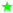:

the parameter values and ranges used to generate the data sets allow for a satisfactory control of the systematic uncertainties;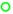:

the parameter values and ranges used to generate the data sets allow for a reasonable attempt at estimating systematic uncertainties, which however could be improved;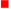:

the parameter values and ranges used to generate the data sets are unlikely to allow for a reasonable control of systematic uncertainties.

The appearance of a red tag, even in a single source of systematic error of a given lattice result, disqualifies it from inclusion in the global average.

Note that in the first two editions [1, 2], FLAG used the three symbols in order to rate the reliability of the systematic errors attributed to a given result by the paper’s authors. Starting with FLAG 16  the meaning of the symbols has changed slightly – they now rate the quality of a particular simulation, based on the values and range of the chosen parameters, and its aptness to obtain well-controlled systematic uncertainties. They do not rate the quality of the analysis performed by the authors of the publication. The latter question is deferred to the relevant sections of the present review, which contain detailed discussions of the results contributing (or not) to each FLAG average or estimate.

For most quantities the colour-coding system refers to the following sources of systematic errors: (i) chiral extrapolation; (ii) continuum extrapolation; (iii) finite volume. As we will see below, renormalization is another source of systematic uncertainties in several quantities. This we also classify using the three coloured symbols listed above, but now with a different rationale: they express how reliably these quantities are renormalized, from a field-theoretic point of view (namely, nonperturbatively, or with 2-loop or 1-loop perturbation theory).

Given the sophisticated status that the field has attained, several aspects, besides those rated by the coloured symbols, need to be evaluated before one can conclude whether a particular analysis leads to results that should be included in an average or estimate. Some of these aspects are not so easily expressible in terms of an adjustable parameter such as the lattice spacing, the pion mass or the volume. As a result of such considerations, it sometimes occurs, albeit rarely, that a given result does not contribute to the FLAG average or estimate, despite not carrying any red tags. This happens, for instance, whenever aspects of the analysis appear to be incomplete (e.g., an incomplete error budget), so that the presence of inadequately controlled systematic effects cannot be excluded. This mostly refers to results with a statistical error only, or results in which the quoted error budget obviously fails to account for an important contribution.

Of course, any colour coding has to be treated with caution; we emphasize that the criteria are subjective and evolving. Sometimes, a single source of systematic error dominates the systematic uncertainty and it is more important to reduce this uncertainty than to aim for green stars for other sources of error. In spite of these caveats, we hope that our attempt to introduce quality measures for lattice simulations will prove to be a useful guide. In addition, we would like to stress that the agreement of lattice results obtained using different actions and procedures provides further validation.

#### 2.1.1 Systematic effects and rating criteria

The precise criteria used in determining the colour coding are unavoidably time-dependent; as lattice calculations become more accurate, the standards against which they are measured become tighter. For this reason FLAG reassesses criteria with each edition and as a result some of the quality criteria (the one on chiral extrapolation for instance) have been tightened up over time [1,2,3,4].

In the following, we present the rating criteria used in the current report. While these criteria apply to most quantities without modification there are cases where they need to be amended or additional criteria need to be defined. For instance, when discussing results obtained in the $$\epsilon$$-regime of chiral perturbation theory in Sect. 5 the finite volume criterion listed below for the p-regime is no longer appropriate.Footnote 4 Similarly, the discussion of the strong coupling constant in Sect. 9 requires tailored criteria for renormalization, perturbative behaviour, and continuum extrapolation. Finally, in the section on nuclear matrix elements, Sect. 10, the chiral extrapolation criterion is made slightly stronger, and a new criterion is adopted for excited-state contributions. In such cases, the modified criteria are discussed in the respective sections. Apart from only a few exceptions the following colour code applies in the tables:

• Chiral extrapolation: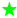:

$$M_{\pi ,\textrm{min}}< 200$$ MeV, with three or more pion masses used in the extrapolation

or two values of $$M_\pi$$ with one lying within 10 MeV of 135 MeV (the physical neutral pion mass) and the other one below 200 MeV:

200 MeV $$\le M_{\pi ,{\textrm{min}}} \le$$ 400 MeV, with three or more pion masses used in the extrapolation

or two values of $$M_\pi$$ with $$M_{\pi ,{\textrm{min}}}<$$ 200 MeV

or a single value of $$M_\pi$$, lying within 10 MeV of 135 MeV (the physical neutral pion mass):

otherwise

This criterion is unchanged from FLAG 19. In Sect. 10 the upper end of the range for $$M_{\pi ,{\textrm{min}}}$$ in the green circle criterion is lowered to 300 MeV, as in FLAG 19.

• Continuum extrapolation::

at least three lattice spacings and at least two points below 0.1 fm and a range of lattice spacings satisfying $$[a_{{\textrm{max}}}/a_{\textrm{min}}]^2 \ge 2$$:

at least two lattice spacings and at least one point below 0.1 fm and a range of lattice spacings satisfying $$[a_{{\textrm{max}}}/a_{\textrm{min}}]^2 \ge 1.4$$:

otherwise

It is assumed that the lattice action is $${\mathcal {O}}(a)$$-improved (i.e., the discretization errors vanish quadratically with the lattice spacing); otherwise this will be explicitly mentioned. For unimproved actions an additional lattice spacing is required. This condition is unchanged from FLAG 19.

• Finite-volume effects:

The finite-volume colour code used for a result is chosen to be the worse of the QCD and the QED codes, as described below. If only QCD is used the QED colour code is ignored. – For QCD:

•$$[M_{\pi ,\textrm{min}} / M_{\pi ,\textrm{fid}}]^2 \exp \{4-M_{\pi ,\textrm{min}}[L(M_{\pi ,\textrm{min}})]_{{\textrm{max}}}\} < 1$$, or at least three volumes

•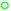$$[M_{\pi ,\textrm{min}} / M_{\pi ,\textrm{fid}}]^2 \exp \{3-M_{\pi ,\textrm{min}}[L(M_{\pi ,\textrm{min}})]_{{\textrm{max}}}\} < 1$$, or at least two volumes

•otherwise

where we have introduced $$[L(M_{\pi ,\textrm{min}})]_{{\textrm{max}}}$$, which is the maximum box size used in the simulations performed at the smallest pion mass $$M_{\pi ,{{\textrm{min}}}}$$, as well as a fiducial pion mass $$M_{\pi ,\textrm{fid}}$$, which we set to 200 MeV (the cutoff value for a green star in the chiral extrapolation). It is assumed here that calculations are in the p-regime of chiral perturbation theory, and that all volumes used exceed 2 fm. The rationale for this condition is as follows. Finite volume effects contain the universal factor $$\exp \{- L~M_\pi \}$$, and if this were the only contribution a criterion based on the values of $$M_{\pi ,\text {min}} L$$ would be appropriate. However, as pion masses decrease, one must also account for the weakening of the pion couplings. In particular, 1-loop chiral perturbation theory  reveals a behaviour proportional to $$M_\pi ^2 \exp \{- L~M_\pi \}$$. Our condition includes this weakening of the coupling, and ensures, for example, that simulations with $$M_{\pi ,\textrm{min}} = 135~\textrm{MeV}$$ and $$L~M_{\pi ,\textrm{min}} = 3.2$$ are rated equivalently to those with $$M_{\pi ,\textrm{min}} = 200~\textrm{MeV}$$ and $$L~M_{\pi ,\textrm{min}} = 4$$. – For QED (where applicable):

•$$1/([M_{\pi ,\textrm{min}}L(M_{\pi ,\textrm{min}})]_{{\textrm{max}}})^{n_{\textrm{min}}}<0.02$$, or at least four volumes

•$$1/([M_{\pi ,\textrm{min}}L(M_{\pi ,\textrm{min}})]_{{\textrm{max}}})^{n_{\textrm{min}}}<0.04$$, or at least three volumes

•otherwise

Because of the infrared-singular structure of QED, electromagnetic finite-volume effects decay only like a power of the inverse spatial extent. In several cases like mass splittings [147, 148] or leptonic decays , the leading corrections are known to be universal, i.e., independent of the structure of the involved hadrons. In such cases, the leading universal effects can be directly subtracted exactly from the lattice data. We denote $$n_{\textrm{min}}$$ the smallest power of $$\frac{1}{L}$$ at which such a subtraction cannot be done. In the widely used finite-volume formulation $$\textrm{QED}_L$$, one always has $$n_{\textrm{min}}\le 3$$ due to the nonlocality of the theory . The QED criteria are used here only in Sect. 3. Both QCD and QED criteria are unchanged from FLAG 19.

• Isospin breaking effects (where applicable):

•all leading isospin breaking effects are included in the lattice calculation

•isospin breaking effects are included using the electro-quenched approximation

•otherwise

This criterion is used for quantities which are breaking isospin symmetry or which can be determined at the sub-percent accuracy where isospin breaking effects, if not included, are expected to be the dominant source of uncertainty. In the current edition, this criterion is only used for the up- and down-quark masses, and related quantities ($$\epsilon$$, $$Q^2$$ and $$R^2$$). The criteria for isospin breaking effects are unchanged from FLAG 19.

• Renormalization (where applicable):

•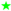nonperturbative

•1-loop perturbation theory or higher with a reasonable estimate of truncation errors

•otherwise

In Ref. , we assigned a red square to all results which were renormalized at 1-loop in perturbation theory. In FLAG 13 , we decided that this was too restrictive, since the error arising from renormalization constants, calculated in perturbation theory at 1-loop, is often estimated conservatively and reliably. These criteria have remained unchanged since then.

• Renormalization Group (RG) running (where applicable):

For scale-dependent quantities, such as quark masses or $$B_K$$, it is essential that contact with continuum perturbation theory can be established. Various different methods are used for this purpose (cf. Appendix A.3 in FLAG 19 ): Regularization-independent Momentum Subtraction (RI/MOM), the Schrödinger functional, and direct comparison with (resummed) perturbation theory. Irrespective of the particular method used, the uncertainty associated with the choice of intermediate renormalization scales in the construction of physical observables must be brought under control. This is best achieved by performing comparisons between nonperturbative and perturbative running over a reasonably broad range of scales. These comparisons were initially only made in the Schrödinger functional approach, but are now also being performed in RI/MOM schemes. We mark the data for which information about nonperturbative running checks is available and give some details, but do not attempt to translate this into a colour code.

The pion mass plays an important role in the criteria relevant for chiral extrapolation and finite volume. For some of the regularizations used, however, it is not a trivial matter to identify this mass. In the case of twisted-mass fermions, discretization effects give rise to a mass difference between charged and neutral pions even when the up- and down-quark masses are equal: the charged pion is found to be the heavier of the two for twisted-mass Wilson fermions (cf. Ref. ). In early works, typically referring to $$N_f=2$$ simulations (e.g., Refs.  and ), chiral extrapolations are based on chiral perturbation theory formulae which do not take these regularization effects into account. After the importance of accounting for isospin breaking when doing chiral fits was shown in Ref. , later works, typically referring to $$N_f=2+1+1$$ simulations, have taken these effects into account . We use $$M_{\pi ^\pm }$$ for $$M_{\pi ,\textrm{min}}$$ in the chiral-extrapolation rating criterion. On the other hand, we identify $$M_{\pi ,\textrm{min}}$$ with the root mean square (RMS) of $$M_{\pi ^+}$$, $$M_{\pi ^-}$$ and $$M_{\pi ^0}$$ in the finite-volume rating criterion.

In the case of staggered fermions, discretization effects give rise to several light states with the quantum numbers of the pion.Footnote 5 The mass splitting among these “taste” partners represents a discretization effect of $${\mathcal {O}}(a^2)$$, which can be significant at large lattice spacings but shrinks as the spacing is reduced. In the discussion of the results obtained with staggered quarks given in the following sections, we assume that these artifacts are under control. We conservatively identify $$M_{\pi ,\textrm{min}}$$ with the root mean square (RMS) average of the masses of all the taste partners, both for chiral-extrapolation and finite-volume criteria.

In some of the simulations, the fermion formulations employed for the valence quarks are different from those used for the sea quarks. Even when the fermion formulations are the same, there are cases where the sea and valence quark masses differ. In such cases, we use the smaller of the valence-valence and valence-sea $$M_{\pi _{{\textrm{min}}}}$$ values in the finite-volume criteria, since either of these channels may give the leading contribution depending on the quantity of interest at the one-loop level of chiral perturbation theory. For the chiral-extrapolation criteria, on the other hand, we use the unitary point, where the sea and valence quark masses are the same, to define $$M_{\pi _{{\textrm{min}}}}$$.

The strong coupling $$\alpha _s$$ is computed in lattice QCD with methods differing substantially from those used in the calculations of the other quantities discussed in this review. Therefore, we have established separate criteria for $$\alpha _s$$ results, which will be discussed in Sect. 9.2.1.

In the section on nuclear matrix elements, Sect. 10, an additional criterion is used. This concerns the level of control over contamination from excited states, which is a more challenging issue for nucleons than for mesons. In response to an improved understanding of the impact of this contamination, the excited-state contamination criterion has been made more stringent compared to that in FLAG 19.

#### 2.1.2 Heavy-quark actions

For the b quark, the discretization of the heavy-quark action follows a very different approach from that used for light flavours. There are several different methods for treating heavy quarks on the lattice, each with its own issues and considerations. Most of these methods use Effective Field Theory (EFT) at some point in the computation, either via direct simulation of the EFT, or by using EFT as a tool to estimate the size of cutoff errors, or by using EFT to extrapolate from the simulated lattice quark masses up to the physical b-quark mass. Because of the use of an EFT, truncation errors must be considered together with discretization errors.

The charm quark lies at an intermediate point between the heavy and light quarks. In our earlier reviews, the calculations involving charm quarks often treated it using one of the approaches adopted for the b quark. Since FLAG 16 , however, most calculations simulate the charm quark using light-quark actions. This has become possible thanks to the increasing availability of dynamical gauge field ensembles with fine lattice spacings. But clearly, when charm quarks are treated relativistically, discretization errors are more severe than those of the corresponding light-quark quantities.

In order to address these complications, the heavy-quark section adds an additional, bipartite, treatment category to the rating system. The purpose of this criterion is to provide a guideline for the level of action and operator improvement needed in each approach to make reliable calculations possible, in principle.

A description of the different approaches to treating heavy quarks on the lattice can be found in Appendix A.1.3 of FLAG 19 . For truncation errors we use HQET power counting throughout, since this review is focused on heavy-quark quantities involving B and D mesons rather than bottomonium or charmonium quantities. Here we describe the criteria for how each approach must be implemented in order to receive an acceptable rating (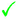) for both the heavy-quark actions and the weak operators. Heavy-quark implementations without the level of improvement described below are rated not acceptable (). The matching is evaluated together with renormalization, using the renormalization criteria described in Sect. 2.1.1. We emphasize that the heavy-quark implementations rated as acceptable and described below have been validated in a variety of ways, such as via phenomenological agreement with experimental measurements, consistency between independent lattice calculations, and numerical studies of truncation errors. These tests are summarized in Sect. 8.

Relativistic heavy-quark actions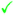at least tree-level $${\mathcal {O}}(a)$$ improved action and weak operators

This is similar to the requirements for light-quark actions. All current implementations of relativistic heavy-quark actions satisfy this criterion.

NRQCDtree-level matched through $${\mathcal {O}}(1/m_h)$$ and improved through $${\mathcal {O}}(a^2)$$

The current implementations of NRQCD satisfy this criterion, and also include tree-level corrections of $${\mathcal {O}}(1/m_h^2)$$ in the action.

HQETtree-level matched through $${\mathcal {O}}(1/m_h)$$ with discretization errors starting at $${\mathcal {O}}(a^2)$$

The current implementation of HQET by the ALPHA collaboration satisfies this criterion, since both action and weak operators are matched nonperturbatively through $${\mathcal {O}}(1/m_h)$$. Calculations that exclusively use a static-limit action do not satisfy this criterion, since the static-limit action, by definition, does not include $$1/m_h$$ terms. We therefore include static computations in our final estimates only if truncation errors (in $$1/m_h$$) are discussed and included in the systematic uncertainties.

Light-quark actions for heavy quarksdiscretization errors starting at $${\mathcal {O}}(a^2)$$ or higher

This applies to calculations that use the twisted-mass Wilson action, a nonperturbatively improved Wilson action, domain wall fermions or the HISQ action for charm-quark quantities. It also applies to calculations that use these light quark actions in the charm region and above together with either the static limit or with an HQET-inspired extrapolation to obtain results at the physical b-quark mass. In these cases, the continuum-extrapolation criteria described earlier must be applied to the entire range of heavy-quark masses used in the calculation.

#### 2.1.3 Conventions for the figures

For a coherent assessment of the present situation, the quality of the data plays a key role, but the colour coding cannot be carried over to the figures. On the other hand, simply showing all data on equal footing might give the misleading impression that the overall consistency of the information available on the lattice is questionable. Therefore, in the figures we indicate the quality of the data in a rudimentary way, using the following symbols: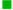:

corresponds to results included in the average or estimate (i.e., results that contribute to the black square below);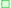:

corresponds to results that are not included in the average but pass all quality criteria;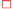:

corresponds to all other results;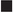:

corresponds to FLAG averages or estimates; they are also highlighted by a gray vertical band.

The reason for not including a given result in the average is not always the same: the result may fail one of the quality criteria; the paper may be unpublished; it may be superseded by newer results; or it may not offer a complete error budget.

Symbols other than squares are used to distinguish results with specific properties and are always explained in the caption.Footnote 6

Often, nonlattice data are also shown in the figures for comparison. For these we use the following symbols: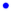:

corresponds to nonlattice results;

$$\blacktriangle$$:

corresponds to Particle Data Group (PDG) results.

### 2.2 Averages and estimates

FLAG results of a given quantity are denoted either as averages or as estimates. Here we clarify this distinction. To start with, both averages and estimates are based on results without any red tags in their colour coding. For many observables there are enough independent lattice calculations of good quality, with all sources of error (not merely those related to the colour-coded criteria), as analyzed in the original papers, appearing to be under control. In such cases, it makes sense to average these results and propose such an average as the best current lattice number. The averaging procedure applied to this data and the way the error is obtained is explained in detail in Sect. 2.3. In those cases where only a sole result passes our rating criteria (colour coding), we refer to it as our FLAG average, provided it also displays adequate control of all other sources of systematic uncertainty.

On the other hand, there are some cases in which this procedure leads to a result that, in our opinion, does not cover all uncertainties. Systematic errors are by their nature often subjective and difficult to estimate, and may thus end up being underestimated in one or more results that receive green symbols for all explicitly tabulated criteria. Adopting a conservative policy, in these cases we opt for an estimate (or a range), which we consider as a fair assessment of the knowledge acquired on the lattice at present. This estimate is not obtained with a prescribed mathematical procedure, but reflects what we consider the best possible analysis of the available information. The hope is that this will encourage more detailed investigations by the lattice community.

There are two other important criteria that also play a role in this respect, but that cannot be colour coded, because a systematic improvement is not possible. These are: (i) the publication status, and (ii) the number of sea-quark flavours $$N_{\,f}$$. As far as the former criterion is concerned, we adopt the following policy: we average only results that have been published in peer-reviewed journals, i.e., they have been endorsed by referee(s). The only exception to this rule consists in straightforward updates of previously published results, typically presented in conference proceedings. Such updates, which supersede the corresponding results in the published papers, are included in the averages. Note that updates of earlier results rely, at least partially, on the same gauge-field-configuration ensembles. For this reason, we do not average updates with earlier results. Nevertheless, all results are listed in the tables,Footnote 7 and their publication status is identified by the following symbols:

• Publication status:

A   published or plain update of published results

P   preprint

C   conference contribution

In the present edition, the publication status on the 30th of April 2021 is relevant. If the paper appeared in print after that date, this is accounted for in the bibliography, but does not affect the averages.Footnote 8

As noted above, in this review we present results from simulations with $$N_f=2$$, $$N_f=2+1$$ and $$N_f=2+1+1$$ (except for $$r_0 \Lambda _{\overline{\textrm{MS}}}$$ where we also give the $$N_f=0$$ result). We are not aware of an a priori way to quantitatively estimate the difference between results produced in simulations with a different number of dynamical quarks. We therefore average results at fixed $$N_{\,f}$$ separately; averages of calculations with different $$N_{\,f}$$ are not provided.

To date, no significant differences between results with different values of $$N_f$$ have been observed in the quantities listed in Tables 1, 2, 3, 4, and 5. In particular, differences between results from simulations with $$N_{\,f}= 2$$ and $$N_{\,f}= 2 + 1$$ would reflect Zweig-rule violations related to strange-quark loops. Although not of direct phenomenological relevance, the size of such violations is an interesting theoretical issue per se, and one that can be quantitatively addressed only with lattice calculations. It remains to be seen whether the status presented here will change in the future, since this will require dedicated $$N_f=2$$ and $$N_f=2+1$$ calculations, which are not a priority of present lattice work.

The question of differences between results with $$N_{\,f}=2+1$$ and $$N_{\,f}=2+1+1$$ is more subtle. The dominant effect of including the charm sea quark is to shift the lattice scale, an effect that is accounted for by fixing this scale nonperturbatively using physical quantities. For most of the quantities discussed in this review, it is expected that residual effects are small in the continuum limit, suppressed by $$\alpha _s(m_c)$$ and powers of $$\Lambda ^2/m_c^2$$. Here $$\Lambda$$ is a hadronic scale that can only be roughly estimated and depends on the process under consideration. Note that the $$\Lambda ^2/m_c^2$$ effects have been addressed in Refs. [158,159,160,161,162], and found to be small for the quantities considered. Assuming that such effects are generically small, it might be reasonable to average the results from $$N_{\,f}=2+1$$ and $$N_{\,f}=2+1+1$$ simulations, although we do not do so here.

### 2.3 Averaging procedure and error analysis

In the present report, we repeatedly average results obtained by different collaborations, and estimate the error on the resulting averages. Here we provide details on how averages are obtained.

#### 2.3.1 Averaging – generic case

We follow the procedure of the previous two editions [2, 3], which we describe here in full detail.

One of the problems arising when forming averages is that not all of the data sets are independent. In particular, the same gauge-field configurations, produced with a given fermion discretization, are often used by different research teams with different valence-quark lattice actions, obtaining results that are not really independent. Our averaging procedure takes such correlations into account.

Consider a given measurable quantity Q, measured by M distinct, not necessarily uncorrelated, numerical experiments (simulations). The result of each of these measurement is expressed as

\begin{aligned} Q_i = x_i \, \pm \, \sigma ^{(1)}_i \pm \, \sigma ^{(2)}_i \pm \cdots \pm \, \sigma ^{(E)}_i, \end{aligned}
(1)

where $$x_i$$ is the value obtained by the ith experiment ($$i = 1, \ldots , M$$) and $$\sigma ^{(\alpha )}_i$$ (for $$\alpha = 1, \ldots , E$$) are the various errors. Typically $$\sigma ^{(1)}_i$$ stands for the statistical error and $$\sigma ^{(\alpha )}_i$$ ($$\alpha \ge 2$$) are the different systematic errors from various sources. For each individual result, we estimate the total error $$\sigma _i$$ by adding statistical and systematic errors in quadrature:

\begin{aligned} Q_i= & {} x_i \, \pm \, \sigma _i, \nonumber \\ \sigma _i\equiv & {} \sqrt{\sum _{\alpha =1}^E \Big [\sigma ^{(\alpha )}_i \Big ]^2}. \end{aligned}
(2)

With the weight factor of each total error estimated in standard fashion,

\begin{aligned} \omega _i = \dfrac{\sigma _i^{-2}}{\sum _{i=1}^M \sigma _i^{-2}}, \end{aligned}
(3)

the central value of the average over all simulations is given by

\begin{aligned} x_\textrm{av}= & {} \sum _{i=1}^M x_i\, \omega _i. \end{aligned}
(4)

The above central value corresponds to a $$\chi _{{\textrm{min}}}^2$$ weighted average, evaluated by adding statistical and systematic errors in quadrature. If the fit is not of good quality ($$\chi _{{\textrm{min}}}^2/\textrm{dof} > 1$$), the statistical and systematic error bars are stretched by a factor $$S = \sqrt{\chi ^2/\textrm{dof}}$$.

Next, we examine error budgets for individual calculations and look for potentially correlated uncertainties. Specific problems encountered in connection with correlations between different data sets are described in the text that accompanies the averaging. If there is reason to believe that a source of error is correlated between two calculations, a $$100\%$$ correlation is assumed. The correlation matrix $$C_{ij}$$ for the set of correlated lattice results is estimated by a prescription due to Schmelling . This consists in defining

\begin{aligned} \sigma _{i;j} = \sqrt{{\sum _{\alpha }}^\prime \Big [ \sigma _i^{(\alpha )} \Big ]^2 }, \end{aligned}
(5)

with $$\sum _{\alpha }^\prime$$ running only over those errors of $$x_i$$ that are correlated with the corresponding errors of the measurement $$x_j$$. This expresses the part of the uncertainty in $$x_i$$ that is correlated with the uncertainty in $$x_j$$. If no such correlations are known to exist, then we take $$\sigma _{i;j} =0$$. The diagonal and off-diagonal elements of the correlation matrix are then taken to be

\begin{aligned} C_{ii}= & {} \sigma _i^2 \qquad \qquad (i = 1, \ldots , M), \nonumber \\ C_{ij}= & {} \sigma _{i;j} \, \sigma _{j;i} \qquad \qquad (i \ne j). \end{aligned}
(6)

Finally, the error of the average is estimated by

\begin{aligned} \sigma ^2_\textrm{av} = \sum _{i=1}^M \sum _{j=1}^M \omega _i \,\omega _j \,C_{ij}, \end{aligned}
(7)

and the FLAG average is

\begin{aligned} Q_\textrm{av} = x_\textrm{av} \, \pm \, \sigma _\textrm{av}. \end{aligned}
(8)

#### 2.3.2 Nested averaging

We have encountered one case where the correlations between results are more involved, and a nested averaging scheme is required. This concerns the B-meson bag parameters discussed in Sect. 8.2. In the following, we describe the details of the nested averaging scheme. This is an updated version of the section added in the web update of the FLAG 16 report.

The issue arises for a quantity Q that is given by a ratio, $$Q=Y/Z$$. In most simulations, both Y and Z are calculated, and the error in Q can be obtained in each simulation in the standard way. However, in other simulations only Y is calculated, with Z taken from a global average of some type. The issue to be addressed is that this average value $$\overline{Z}$$ has errors that are correlated with those in Q.

In the example that arises in Sect. 8.2, $$Q=B_B$$, $$Y=B_B f_B^2$$ and $$Z=f_B^2$$. In one of the simulations that contribute to the average, Z is replaced by $$\overline{Z}$$, the PDG average for $$f_B^2$$  (obtained with an averaging procedure similar to that used by FLAG). This simulation is labeled with $$i=1$$, so that

\begin{aligned} Q_1 = \frac{Y_1}{\overline{Z}}. \end{aligned}
(9)

The other simulations have results labeled $$Q_j$$, with $$j\ge 2$$. In this set up, the issue is that $$\overline{Z}$$ is correlated with the $$Q_j$$, $$j\ge 2$$.Footnote 9

We begin by decomposing the error in $$Q_1$$ in the same schematic form as above,

\begin{aligned} Q_1 = x_1 \pm \frac{\sigma _{Y_1}^{(1)}}{\overline{Z}} \pm \frac{\sigma _{Y_1}^{(2)}}{\overline{Z}} \pm \cdots \pm \frac{\sigma _{Y_1}^{(E)}}{\overline{Z}} \pm \frac{Y_1 \sigma _{\overline{Z}}}{\overline{Z}^2}. \end{aligned}
(10)

Here the last term represents the error propagating from that in $$\overline{Z}$$, while the others arise from errors in $$Y_1$$. For the remaining $$Q_j$$ ($$j\ge 2$$) the decomposition is as in Eq. (1). The total error of $$Q_1$$ then reads

\begin{aligned} \sigma _1^2 = \left( \frac{\sigma _{Y_1}^{(1)}}{\overline{Z}}\right) ^2 + \left( \frac{\sigma _{Y_1}^{(2)}}{\overline{Z}}\right) ^2 +\cdots + \left( \frac{\sigma _{Y_1}^{(E)}}{\overline{Z}}\right) ^2 + \left( \frac{Y_1}{\overline{Z}^2}\right) ^2 \sigma _{\overline{Z}}^2, \end{aligned}
(11)

while that for the $$Q_j$$ ($$j\ge 2$$) is

\begin{aligned} \sigma _j^2 = \left( \sigma _j^{(1)}\right) ^2 + \left( \sigma _j^{(2)}\right) ^2 +\cdots + \left( \sigma _j^{(E)}\right) ^2. \end{aligned}
(12)

Correlations between $$Q_j$$ and $$Q_k$$ ($$j,k\ge 2$$) are taken care of by Schmelling’s prescription, as explained above. What is new here is how the correlations between $$Q_1$$ and $$Q_j$$ ($$j\ge 2$$) are taken into account.

To proceed, we recall from Eq. (7) that $$\sigma _{\overline{Z}}$$ is given by

\begin{aligned} \sigma _{\overline{Z}}^2 = \sum _{{i'},{j'}=1}^{M'} \omega [Z]_{i'} \omega [Z]_{j'} C[Z]_{i'j'}. \end{aligned}
(13)

Here the indices $$i'$$ and $$j'$$ run over the $$M'$$ simulations that contribute to $$\overline{Z}$$, which, in general, are different from those contributing to the results for Q. The weights $$\omega [Z]$$ and correlation matrix C[Z] are given an explicit argument Z to emphasize that they refer to the calculation of this quantity and not to that of Q. C[Z] is calculated using the Schmelling prescription [Eqs. (5)–(7)] in terms of the errors, $$\sigma [Z]_{i'}^{(\alpha )}$$, taking into account the correlations between the different calculations of Z.

We now generalize Schmelling’s prescription for $$\sigma _{i;j}$$, Eq. (5), to that for $$\sigma _{1;k}$$ ($$k\ge 2$$), i.e., the part of the error in $$Q_1$$ that is correlated with $$Q_k$$. We take

\begin{aligned} \sigma _{1;k} = \sqrt{ \frac{1}{\overline{Z}^2} \sum ^\prime _{(\alpha )\leftrightarrow k} \Big [\sigma _{Y_1}^{(\alpha )} \Big ]^2 + \frac{Y_1^2}{\overline{Z}^4} \sum _{i',j'}^{M'} \omega [Z]_{i'} \omega [Z]_{j'} C[Z]_{i'j'\leftrightarrow k} }. \end{aligned}
(14)

The first term under the square root sums those sources of error in $$Y_1$$ that are correlated with $$Q_k$$. Here we are using a more explicit notation from that in Eq. (5), with $$(\alpha ) \leftrightarrow k$$ indicating that the sum is restricted to the values of $$\alpha$$ for which the error $$\sigma _{Y_1}^{(\alpha )}$$ is correlated with $$Q_k$$. The second term accounts for the correlations within $$\overline{Z}$$ with $$Q_k$$, and is the nested part of the present scheme. The new matrix $$C[Z]_{i'j'\leftrightarrow k}$$ is a restriction of the full correlation matrix C[Z], and is defined as follows. Its diagonal elements are given by

\begin{aligned} C[Z]_{i'i'\leftrightarrow k}= & {} (\sigma [Z]_{i'\leftrightarrow k})^2 \qquad \qquad (i' = 1, \ldots , M'), \end{aligned}
(15)
\begin{aligned} (\sigma [Z]_{i'\leftrightarrow k})^2= & {} \sum ^\prime _{(\alpha )\leftrightarrow k} (\sigma [Z]_{i'}^{(\alpha )})^2, \end{aligned}
(16)

where the summation $$\sum ^\prime _{(\alpha )\leftrightarrow k}$$ over $$(\alpha )$$ is restricted to those $$\sigma [Z]_{i'}^{(\alpha )}$$ that are correlated with $$Q_k$$. The off-diagonal elements are

\begin{aligned} C[Z]_{i'j'\leftrightarrow k}= & {} \sigma [Z]_{i';j'\leftrightarrow k} \, \sigma [Z]_{j';i'\leftrightarrow k} \qquad \qquad (i' \ne j'), \end{aligned}
(17)
\begin{aligned} \sigma [Z]_{i';j'\leftrightarrow k}= & {} \sqrt{ \sum ^\prime _{(\alpha )\leftrightarrow j'k} (\sigma [Z]_{i'}^{(\alpha )})^2}, \end{aligned}
(18)

where the summation $$\sum ^\prime _{(\alpha )\leftrightarrow j'k}$$ over $$(\alpha )$$ is restricted to $$\sigma [Z]_{i'}^{(\alpha )}$$ that are correlated with both $$Z_{j'}$$ and $$Q_k$$.

The last quantity that we need to define is $$\sigma _{k;1}$$.

\begin{aligned} \sigma _{k;1} = \sqrt{\sum ^\prime _{(\alpha )\leftrightarrow 1} \Big [ \sigma _k^{(\alpha )} \Big ]^2 }, \end{aligned}
(19)

where the summation $$\sum ^\prime _{(\alpha )\leftrightarrow 1}$$ is restricted to those $$\sigma _k^{(\alpha )}$$ that are correlated with one of the terms in Eq. (11).

In summary, we construct the correlation matrix $$C_{ij}$$ using Eq. (6), as in the generic case, except the expressions for $$\sigma _{1;k}$$ and $$\sigma _{k;1}$$ are now given by Eqs. (14) and (19), respectively. All other $$\sigma _{i;j}$$ are given by the original Schmelling prescription, Eq. (5). In this way we extend the philosophy of Schmelling’s approach while accounting for the more involved correlations.

## 3 Quark masses

Authors: T. Blum, A. Portelli, A. Ramos

Quark masses are fundamental parameters of the Standard Model. An accurate determination of these parameters is important for both phenomenological and theoretical applications. The bottom- and charm-quark masses, for instance, are important sources of parametric uncertainties in several Higgs decay modes. The up-, down- and strange-quark masses govern the amount of explicit chiral symmetry breaking in QCD. From a theoretical point of view, the values of quark masses provide information about the flavour structure of physics beyond the Standard Model. The Review of Particle Physics of the Particle Data Group contains a review of quark masses , which covers light as well as heavy flavours. Here we also consider light- and heavy-quark masses, but focus on lattice results and discuss them in more detail. We do not discuss the top quark, however, because it decays weakly before it can hadronize, and the nonperturbative QCD dynamics described by present day lattice simulations is not relevant. The lattice determination of light- (up, down, strange), charm- and bottom-quark masses is considered below in Sects. 3.1, 3.2, and 3.3, respectively.

Quark masses cannot be measured directly in experiment because quarks cannot be isolated, as they are confined inside hadrons. From a theoretical point of view, in QCD with $$N_f$$ flavours, a precise definition of quark masses requires one to choose a particular renormalization scheme. This renormalization procedure introduces a renormalization scale $$\mu$$, and quark masses depend on this renormalization scale according to the Renormalization Group (RG) equations. In mass-independent renormalization schemes the RG equations read

\begin{aligned} \mu \frac{\textrm{d} {\bar{m}}_i(\mu )}{\textrm{d}{\mu }} = {\bar{m}}_i(\mu ) \tau ({\bar{g}}), \end{aligned}
(20)

where the function $$\tau ({\bar{g}})$$ is the anomalous dimension, which depends only on the value of the strong coupling $$\alpha _s={\bar{g}}^2/(4\pi )$$. Note that in QCD $$\tau ({\bar{g}})$$ is the same for all quark flavours. The anomalous dimension is scheme dependent, but its perturbative expansion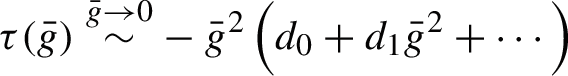(21)

has a leading coefficient $$d_0 = 8/(4\pi )^2$$, which is scheme independent.Footnote 10 Equation (20), being a first order differential equation, can be solved exactly by using Eq. (21) as the boundary condition. The formal solution of the RG equation reads

\begin{aligned} M_i = {\bar{m}}_i(\mu )[2b_0{\bar{g}}^2(\mu )]^{-d_0/(2b_0)} \exp \left\{ - \int _0^{{\bar{g}}(\mu )}\textrm{d} x\, \left[ \frac{\tau (x)}{\beta (x)} - \frac{d_0}{b_0x} \right] \right\} , \end{aligned}
(22)

where $$b_0 = (11-2N_f/3) / (4\pi )^2$$ is the universal leading perturbative coefficient in the expansion of the $$\beta$$-function, $$\beta (\bar{g}) \equiv d\bar{g}^2/d\log \mu ^2$$, which governs the running of the strong coupling constant near the scale $$\mu$$. The renormalization group invariant (RGI) quark masses $$M_i$$ are formally integration constants of the RG Eq. (20). They are scale independent, and due to the universality of the coefficient $$d_0$$, they are also scheme independent. Moreover, they are nonperturbatively defined by Eq. (22). They only depend on the number of flavours $$N_f$$, making them a natural candidate to quote quark masses and compare determinations from different lattice collaborations. Nevertheless, it is customary in the phenomenology community to use the $$\overline{\textrm{MS}}$$ scheme at a scale $$\mu = 2$$ GeV to compare different results for light-quark masses, and use a scale equal to its own mass for the charm and bottom quarks. In this review, we will quote the final averages of both quantities.

Results for quark masses are always quoted in the four-flavour theory. $$N_{f}=2+1$$ results have to be converted to the four-flavour theory. Fortunately, the charm quark is heavy $$(\Lambda _\textrm{QCD}/m_c)^2<1$$, and this conversion can be performed in perturbation theory with negligible ($$\sim 0.2\%$$) perturbative uncertainties. Nonperturbative corrections in this matching are more difficult to estimate. Since these effects are suppressed by a factor of $$1/N_\textrm{c}$$, and a factor of the strong coupling at the scale of the charm mass, naive power counting arguments would suggest that the effects are $$\sim 1\%$$. In practice, numerical nonperturbative studies [158, 160, 167] have found this power counting argument to be an overestimate by one order of magnitude in the determination of simple hadronic quantities or the $$\Lambda$$-parameter. Moreover, lattice determinations do not show any significant deviation between the $$N_{f}=2+1$$ and $$N_{f}=2+1+1$$ simulations. For example, the difference in the final averages for the mass of the strange quark $$m_s$$ between $$N_f=2+1$$ and $$N_f=2+1+1$$ determinations is about 1.3%, or about one standard deviation.

We quote all final averages at 2 GeV in the $$\overline{\textrm{MS}}$$ scheme and also the RGI values (in the four-flavour theory). We use the exact RG Eq. (22). Note that to use this equation we need the value of the strong coupling in the $$\overline{\textrm{MS}}$$ scheme at a scale $$\mu = 2$$ GeV. All our results are obtained from the RG equation in the $$\overline{\textrm{MS}}$$ scheme and the 5-loop beta function together with the value of the $$\Lambda$$-parameter in the four-flavour theory $$\Lambda ^{(4)}_{\overline{\textrm{MS}}} = 294(12)\, \textrm{MeV}$$ obtained in this review (see Sect. 9). In the uncertainties of the RGI masses we separate the contributions from the determination of the quark masses and the propagation of the uncertainty of $$\Lambda ^{(4)}_{\overline{\textrm{MS}}}$$. These are identified with the subscripts m and $$\Lambda$$, respectively.

Conceptually, all lattice determinations of quark masses contain three basic ingredients:

1. 1.

Tuning the lattice bare-quark masses to match the experimental values of some quantities. Pseudo-scalar meson masses provide the most common choice, since they have a strong dependence on the values of quark masses. In pure QCD with $$N_f$$ quark flavours these values are not known, since the electromagnetic interactions affect the experimental values of meson masses. Therefore, pure QCD determinations use model/lattice information to determine the location of the physical point. This is discussed at length in Sect. 3.1.1.

2. 2.

Renormalization of the bare-quark masses. Bare-quark masses determined with the above-mentioned criteria have to be renormalized. Many of the latest determinations use some nonperturbatively defined scheme. One can also use perturbation theory to connect directly the values of the bare-quark masses to the values in the $$\overline{\textrm{MS}}$$ scheme at 2 GeV. Experience shows that 1-loop calculations are unreliable for the renormalization of quark masses: usually at least two loops are required to have trustworthy results.

3. 3.

If quark masses have been nonperturbatively renormalized, for example, to some MOM/SF scheme, the values in this scheme must be converted to the phenomenologically useful values in the $$\overline{\textrm{MS}}$$ scheme (or to the scheme/scale independent RGI masses). Either option requires the use of perturbation theory. The larger the energy scale of this matching with perturbation theory, the better, and many recent computations in MOM schemes do a nonperturbative running up to 3–4 GeV. Computations in the SF scheme allow us to perform this running nonperturbatively over large energy scales and match with perturbation theory directly at the electro-weak scale $$\sim 100$$ GeV.

Note that many lattice determinations of quark masses make use of perturbation theory at a scale of a few GeV.

We mention that lattice-QCD calculations of the b-quark mass have an additional complication which is not present in the case of the charm and light quarks. At the lattice spacings currently used in numerical simulations the direct treatment of the b quark with the fermionic actions commonly used for light quarks is very challenging. Only two determinations of the b-quark mass use this approach, reaching the physical b-quark mass region at two lattice spacings with $$aM\sim 1$$. There are a few widely used approaches to treat the b quark on the lattice, which have been already discussed in the FLAG 13 review (see Sec. 8 of Ref. ). Those relevant for the determination of the b-quark mass will be briefly described in Sect. 3.3.

### 3.1 Masses of the light quarks

Light-quark masses are particularly difficult to determine because they are very small (for the up and down quarks) or small (for the strange quark) compared to typical hadronic scales. Thus, their impact on typical hadronic observables is minute, and it is difficult to isolate their contribution accurately.

Fortunately, the spontaneous breaking of $$SU(3)_L\times SU(3)_R$$ chiral symmetry provides observables which are particularly sensitive to the light-quark masses: the masses of the resulting Nambu–Goldstone bosons (NGB), i.e., pions, kaons, and eta. Indeed, the Gell–Mann–Oakes–Renner relation  predicts that the squared mass of a NGB is directly proportional to the sum of the masses of the quark and antiquark which compose it, up to higher-order mass corrections. Moreover, because these NGBs are light, and are composed of only two valence particles, their masses have a particularly clean statistical signal in lattice-QCD calculations. In addition, the experimental uncertainties on these meson masses are negligible. Thus, in lattice calculations, light-quark masses are typically obtained by renormalizing the input quark mass and tuning them to reproduce NGB masses, as described above.

#### 3.1.1 The physical point and isospin symmetry

As mentioned in Sect. 2.1, the present review relies on the hypothesis that, at low energies, the Lagrangiandescribes nature to a high degree of precision. However, most of the results presented below are obtained in pure QCD calculations, which do not include QED. Quite generally, when comparing QCD calculations with experiment, radiative corrections need to be applied. In pure QCD simulations, where the parameters are fixed in terms of the masses of some of the hadrons, the electromagnetic contributions to these masses must be discussed. How the matching is done is generally ambiguous because it relies on the unphysical separation of QCD and QED contributions. In this section, and in the following, we discuss this issue in detail. A related discussion, in the context of scale setting, is given in Sect. 11.3. Of course, once QED is included in lattice calculations, the subtraction of electromagnetic contributions is no longer necessary.

Let us start from the unambiguous case of QCD+QED. As explained in the introduction of this section, the physical quark masses are the parameters of the Lagrangian such that a given set of experimentally measured, dimensionful hadronic quantities are reproduced by the theory. Many choices are possible for these quantities, but in practice many lattice groups use pseudoscalar meson masses, as they are easily and precisely obtained both by experiment, and through lattice simulations. For example, in the four-flavour case, one can solve the system

\begin{aligned} M_{\pi ^+}(m_u,m_d,m_s,m_c,\alpha )= & {} M_{\pi ^+}^{\mathrm {exp.}}\,, \end{aligned}
(23)
\begin{aligned} M_{K^+}(m_u,m_d,m_s,m_c,\alpha )= & {} M_{K^+}^{\mathrm {exp.}}\,, \end{aligned}
(24)
\begin{aligned} M_{K^0}(m_u,m_d,m_s,m_c,\alpha )= & {} M_{K^0}^{\mathrm {exp.}}\,, \end{aligned}
(25)
\begin{aligned} M_{D^0}(m_u,m_d,m_s,m_c,\alpha )= & {} M_{D^0}^{\mathrm {exp.}}\,, \end{aligned}
(26)

where we assumed that

• all the equations are in the continuum and infinite-volume limits;

• the overall scale has been set to its physical value, generally through some lattice-scale setting procedure involving a fifth dimensionful input (see the discussion in Sect. 11.3);

• the quark masses $$m_q$$ are assumed to be renormalized from the bare, lattice ones in some given continuum renormalization scheme;

• $$\alpha =\frac{e^2}{4\pi }$$ is the fine-structure constant expressed as function of the positron charge e, generally set to the Thomson limit $$\alpha =0.007297352\dots$$ ;

• the mass $$M_{h}(m_u,m_d,m_s,m_c,\alpha )$$ of the meson h is a function of the quark masses and $$\alpha$$. The functional dependence is generally obtained by choosing an appropriate parameterization and performing a global fit to the lattice data;

• the superscript exp. indicates that the mass is an experimental input, lattice groups use in general the values in the Particle Data Group review .

However, ambiguities arise with simulations of QCD only. In that case, there is no experimentally measurable quantity that emerges from the strong interaction only. The missing QED contribution is tightly related to isospin-symmetry breaking effects. Isospin symmetry is explicitly broken by the differences between the up- and down-quark masses $$\delta m=m_u-m_d$$, and electric charges $$\delta Q=Q_u-Q_d$$. These effects are, respectively, of order $${\mathcal {O}}(\delta m/\Lambda _{\textrm{QCD}})$$ and $${\mathcal {O}}(\alpha )$$, and are expected to be $${\mathcal {O}}(1\%)$$ of a typical isospin-symmetric hadronic quantity. Strong and electromagnetic isospin-breaking effects are of the same order and therefore cannot, in principle, be evaluated separately without introducing strong ambiguities. Because these effects are small, they can be treated as a perturbation,

\begin{aligned} X(m_u,m_d,m_s,m_c,\alpha )=\bar{X}(m_{ud}, m_s, m_c) +\delta mA_X(m_{ud}, m_s, m_c) +\alpha B_X(m_{ud}, m_s, m_c)\,, \end{aligned}
(27)

for a given hadronic quantity X, where $$m_{ud}=\frac{1}{2}(m_u+m_d)$$ is the average light-quark mass. There are several things to notice here. Firstly, the neglected higher-order $${\mathcal {O}}(\delta m^2,\alpha \delta m,\alpha ^2)$$ corrections are expected to be $${\mathcal {O}}(10^{-4})$$ relatively to X, which at the moment is way beyond the relative statistical accuracy that can be delivered by a lattice calculation. Secondly, this is not strictly speaking an expansion around the isospin-symmetric point, the electromagnetic interaction has also symmetric contributions. From this last expression the previous statements about ambiguities become clearer. Indeed, the only unambiguous prediction one can perform is to solve Eqs. (23)–(26) and use the resulting parameters to obtain a prediction for X, which is represented by the left-hand side of Eq. (27). This prediction will be the sum of the QCD isospin-symmetric part $$\bar{X}$$, the strong isospin-breaking effects $$X^{SU(2)}=\delta mA_X$$, and the electromagnetic effects $$X^{\gamma }=\alpha B_X$$. Obtaining any of these terms individually requires extra, unphysical conditions to perform the separation. To be consistent with previous editions of FLAG, we also define $$\hat{X}=\bar{X}+X^{SU(2)}$$ to be the $$\alpha \rightarrow 0$$ limit of X.

With pure QCD simulations, one typically solves Eqs. (23)–(26) by equating the QCD isospin-symmetric part of a hadron mass $$\bar{M}_h$$, result of the simulations, with its experimental value $$M_h^{\mathrm {exp.}}$$. This will result in an $${\mathcal {O}}(\delta m,\alpha )$$ mis-tuning of the theory parameters which will propagate as an error on predicted quantities. Because of this, in general, one cannot predict hadronic quantities with a relative accuracy higher than $${\mathcal {O}}(1\%)$$ from pure QCD simulations, independently on how the target X is sensitive to isospin-breaking effects. If one performs a complete lattice prediction of the physical value of X, it can be of phenomenological interest to define in some way $$\bar{X}$$, $$X^{SU(2)}$$, and $$X^{\gamma }$$. If we keep $$m_{ud}$$, $$m_s$$ and $$m_c$$ at their physical values in physical units, for a given renormalization scheme and scale, then these three quantities can be extracted by setting successively and simultaneously $$\alpha$$ and $$\delta m$$ to 0. This is where the ambiguity lies: in general the $$\delta m=0$$ point will depend on the renormalization scheme used for the quark masses. In the next section, we give more details on that particular aspect and discuss the order of scheme ambiguities.

#### 3.1.2 Ambiguities in the separation of isospin-breaking contributions

In this section, we discuss the ambiguities that arise in the individual determination of the QED contribution $$X^{\gamma }$$ and the strong-isospin correction $$X^{SU(2)}$$ defined in the previous section. Throughout this section, we assume that the isospin-symmetric quark masses $$m_{ud}$$, $$m_s$$ and $$m_c$$ are always kept fixed in physical units to the values they take at the QCD+QED physical point in some given renormalization scheme. Let us assume that both up and down masses have been renormalized in an identical mass-independent scheme which depends on some energy scale $$\mu$$. We also assume that the renormalization procedure respects chiral symmetry so that quark masses renormalize multiplicatively. The renormalization constants of the quark masses are identical for $$\alpha =0$$ and therefore the renormalized mass of a quark has the general form

\begin{aligned} m_q(\mu )=Z_m(\mu )[1+\alpha Q_{\mathrm {tot.}}^2\delta _{Z}^{(0)}(\mu ) +\alpha Q_{\mathrm {tot.}}Q_q\delta _{Z}^{(1)}(\mu ) +\alpha Q_q^2\delta _{Z}^{(2)}(\mu ) ]m_{q,0} \,, \end{aligned}
(28)

up to $${\mathcal {O}}(\alpha ^2)$$ corrections, where $$m_{q,0}$$ is the bare-quark mass, $$Q_{\mathrm {tot.}}$$ and $$Q_{\mathrm {tot.}}^2$$ are the sum of all quark charges and squared charges, respectively, and $$Q_q$$ is the quark charge, all in units of in units of the positron charge e. Throughout this section, a subscript ud generally denotes the average between up and down quantities and $$\delta$$ the difference between the up and the down quantities. The source of the ambiguities described in the previous section is the mixing of the isospin-symmetric mass $$m_{ud}$$ and the difference $$\delta m$$ through renormalization. Using Eq. (28) one can make this mixing explicit at leading order in $$\alpha$$:

\begin{aligned} \begin{pmatrix} m_{ud}(\mu )\\ \delta m(\mu )\end{pmatrix} =Z_m(\mu )[1+\alpha Q_{\mathrm {tot.}} ^2\delta _{Z}^{(0)}(\mu )+\alpha M^{(1)}(\mu )+\alpha M^{(2)}(\mu )] \begin{pmatrix}m_{ud,0}\\ \delta m_0\end{pmatrix} \end{aligned}
(29)

with the mixing matrices

\begin{aligned} M^{(1)}(\mu )=\delta _Z^{(1)}(\mu )Q_{\mathrm {tot.}} \begin{pmatrix} Q_{ud} &{}\quad \frac{1}{4}\delta Q\\ \delta Q &{}\quad Q_{ud} \end{pmatrix} \qquad \text {and}\qquad M^{(2)}(\mu )=\delta _Z^{(2)}(\mu ) \begin{pmatrix} Q_{ud}^2 &{} \quad \frac{1}{4}\delta Q^2\\ \delta Q^2 &{}\quad Q_{ud}^2 \end{pmatrix}, \end{aligned}
(30)

where $$Q_{ud}=\frac{1}{2}(Q_u+Q_d)$$ and $$\delta Q=Q_u-Q_d$$ are the average and difference of the up and down charges, and similarly $$Q_{ud}^2=\frac{1}{2}(Q_u^2+Q_d^2)$$ and $$\delta Q^2=Q_u^2-Q_d^2$$ for the squared charges. Now let us assume that for the purpose of determining the different components in Eq. (27), one starts by tuning the bare masses to obtain equal up and down masses, for some small coupling $$\alpha _0$$ at some scale $$\mu _0$$, i.e., $$\delta m(\mu _0)=0$$. At this specific point, one can extract the pure QCD, and the QED corrections to a given quantity X by studying the slope of $$\alpha$$ in Eq. (27). From these quantities the strong-isospin contribution can then readily be extracted using a nonzero value of $$\delta m(\mu _0)$$. However, if now the procedure is repeated at another coupling $$\alpha$$ and scale $$\mu$$ with the same bare masses, it appears from Eq. (29) that $$\delta m(\mu )\ne 0$$. More explicitly,

\begin{aligned} \delta m(\mu )=m_{ud}(\mu _0)\frac{Z_m(\mu )}{Z_m(\mu _0)} [\alpha \Delta _Z(\mu ) -\alpha _0\Delta _Z(\mu _0)]\,, \end{aligned}
(31)

with

\begin{aligned} \Delta _Z(\mu )=Q_{\mathrm {tot.}}\delta Q\delta _Z^{(1)}(\mu )+\delta Q^2\delta _Z^{(2)}(\mu )\,,\end{aligned}
(32)

up to higher-order corrections in $$\alpha$$ and $$\alpha _0$$. In other words, the definitions of $$\bar{X}$$, $$X^{SU(2)}$$, and $$X^{\gamma }$$ depend on the renormalization scale at which the separation was made. This dependence, of course, has to cancel in the physical sum X. One can notice that at no point did we mention the renormalization of $$\alpha$$ itself, which, in principle, introduces similar ambiguities. However, the corrections coming from the running of $$\alpha$$ are $${\mathcal {O}}(\alpha ^2)$$ relatively to X, which, as justified above, can be safely neglected. Finally, important information is provided by Eq. (31): the scale ambiguities are $${\mathcal {O}}(\alpha m_{ud})$$. For physical quark masses, one generally has $$m_{ud}\simeq \delta m$$. So by using this approximation in the first-order expansion Eq. (27), it is actually possible to define unambiguously the components of X up to second-order isospin-breaking corrections. Therefore, in the rest of this review, we will not keep track of the ambiguities in determining pure QCD or QED quantities. However, in the context of lattice simulations, it is crucial to notice that $$m_{ud}\simeq \delta m$$ is only accurate at the physical point. In simulations at larger-than-physical pion masses, scheme ambiguities in the separation of QCD and QED contributions are generally large. Once more, the argument made here assumes that the isospin-symmetric quark masses $$m_{ud}$$, $$m_s$$, and $$m_c$$ are kept fixed to their physical value in a given scheme while varying $$\alpha$$. Outside of this assumption there is an additional isospin-symmetric $${\mathcal {O}}(\alpha m_q)$$ ambiguity between $$\bar{X}$$ and $$X^{\gamma }$$.

Such separation in lattice QCD+QED simulation results appeared for the first time in RBC 07  and Blum 10 , where the scheme was implicitly defined around the $$\chi$$PT expansion. In that setup, the $$\delta m(\mu _0)=0$$ point is defined in pure QCD, i.e., $$\alpha _0=0$$ in the previous discussion. The QCD part of the kaon-mass splitting from the first FLAG review  is used as an input in RM123 11 , which focuses on QCD isospin corrections only. It therefore inherits from the convention that was chosen there, which is also to set $$\delta m(\mu _0)=0$$ at zero QED coupling. The same convention was used in the follow-up works RM123 13  and RM123 17 . The BMW collaboration was the first to introduce a purely hadronic scheme in its electro-quenched study of the baryon octet mass splittings . In this work, the quark mass difference $$\delta m(\mu )$$ is swapped with the mass splitting $$\Delta M^2$$ between the connected $$\bar{u}u$$ and $$\bar{d}d$$ pseudoscalar masses. Although unphysical, this quantity is proportional  to $$\delta m(\mu )$$ up to $${\mathcal {O}}(\alpha m_{ud})$$ chiral corrections. In this scheme, the quark masses are assumed to be equal at $$\Delta M^2=0$$, and the $${\mathcal {O}}(\alpha m_{ud})$$ corrections to this statement are analogous to the scale ambiguities mentioned previously. The same scheme was used for the determination of light-quark masses in BMW 16  and in the recent BMW prediction of the leading hadronic contribution to the muon magnetic moment . The BMW collaboration used a different hadronic scheme for its determination of the nucleon-mass splitting in BMW 14  using full QCD+QED simulations. In this work, the $$\delta m=0$$ point was fixed by imposing the baryon splitting $$M_{\Sigma ^+}-M_{\Sigma ^-}$$ to cancel. This scheme is quite different from the other ones presented here, in the sense that its intrinsic ambiguity is not $${\mathcal {O}}(\alpha m_{ud})$$. What motivates this choice here is that $$M_{\Sigma ^+}-M_{\Sigma ^-}=0$$ in the limit where these baryons are point particles, so the scheme ambiguity is suppressed by the compositeness of the $$\Sigma$$ baryons. This may sound like a more difficult ambiguity to quantify, but this scheme has the advantage of being defined purely by measurable quantities. Moreover, it has been demonstrated numerically in BMW 14  that, within the uncertainties of this study, the $$M_{\Sigma ^+}-M_{\Sigma ^-}=0$$ scheme is equivalent to the $$\Delta M^2=0$$ one, explicitly $$M_{\Sigma ^+}-M_{\Sigma ^-}=-0.18(12)(6)\,{\textrm{MeV}}$$ at $$\Delta M^2=0$$. The calculation QCDSF/UKQCD 15  uses a “Dashen scheme,” where quark masses are tuned such that flavour-diagonal mesons have equal masses in QCD and QCD+QED. Although not explicitly mentioned by the authors of the paper, this scheme is simply a reformulation of the $$\Delta M^2=0$$ scheme mentioned previously. Finally, MILC 18  also used the $$\Delta M^2=0$$ scheme and noticed its connection to the “Dashen scheme” from QCDSF/UKQCD 15.

Before the previous edition of this review, the contributions $$\bar{X}$$, $$X^{SU(2)}$$, and $$X^{\gamma }$$ were given for pion and kaon masses based on phenomenological information. Considerable progress has been achieved by the lattice community to include isospin-breaking effects in calculations, and it is now possible to determine these quantities precisely directly from a lattice calculation. However, these quantities generally appear as intermediate products of a lattice analysis, and are rarely directly communicated in publications. These quantities, although unphysical, have a phenomenological interest, and we encourage the authors of future calculations to quote them explicitly.

#### 3.1.3 Inclusion of electromagnetic effects in lattice-QCD simulations

Electromagnetism on a lattice can be formulated using a naive discretization of the Maxwell action $$S[A_{\mu }]=\frac{1}{4}\int d^4 x\,\sum _{\mu ,\nu }[\partial _{\mu }A_{\nu }(x)-\partial _{\nu }A_{\mu }(x)]^2$$. Even in its noncompact form, the action remains gauge invariant. This is not the case for non-Abelian theories for which one uses the traditional compact Wilson gauge action (or an improved version of it). Compact actions for QED feature spurious photon-photon interactions which vanish only in the continuum limit. This is one of the main reason why the noncompact action is the most popular so far. It was used in all the calculations presented in this review. Gauge-fixing is necessary for noncompact actions because of the usual infinite measure of equivalent gauge orbits which contribute to the path integral. It was shown [176, 177] that gauge-fixing is not necessary with compact actions, including in the construction of interpolating operators for charged states.

Although discretization is straightforward, simulating QED in a finite volume is more challenging. Indeed, the long range nature of the interaction suggests that important finite-size effects have to be expected. In the case of periodic boundary conditions, the situation is even more critical: a naive implementation of the theory features an isolated zero-mode singularity in the photon propagator. It was first proposed in  to fix the global zero-mode of the photon field $$A_{\mu }(x)$$ in order to remove it from the dynamics. This modified theory is generally named $$\textrm{QED}_{\textrm{TL}}$$. Although this procedure regularizes the theory and has the right classical infinite-volume limit, it is nonlocal because of the zero-mode fixing. As first discussed in , the nonlocality in time of $$\textrm{QED}_{\textrm{TL}}$$ prevents the existence of a transfer matrix, and therefore a quantum-mechanical interpretation of the theory. Another prescription named $$\textrm{QED}_{\textrm{L}}$$, proposed in , is to remove the zero-mode of $$A_{\mu }(x)$$ independently for each time slice. This theory, although still nonlocal in space, is local in time and has a well-defined transfer matrix. Whether these nonlocalities constitute an issue to extract infinite-volume physics from lattice-QCD+$$\textrm{QED}_{\textrm{L}}$$ simulations is, at the time of this review, still an open question. However, it is known through analytical calculations of electromagnetic finite-size effects at $${\mathcal {O}}(\alpha )$$ in hadron masses [147, 148, 150, 172, 179,180,181], meson leptonic decays , and the hadronic vacuum polarization  that $$\textrm{QED}_{\textrm{L}}$$ does not suffer from a problematic (e.g., UV divergent) coupling of short- and long-distance physics due to its nonlocality. Another strategy, first proposed in  and used by the QCDSF collaboration, is to bound the zero-mode fluctuations to a finite range. Although more minimal, it is still a nonlocal modification of the theory and so far finite-size effects for this scheme have not been investigated. More recently, two proposals for local formulations of finite-volume QED emerged. The first one described in  proposes to use massive photons to regulate zero-mode singularities, at the price of (softly) breaking gauge invariance. The second one presented in , based on earlier works [185, 186], avoids the zero-mode issue by using anti-periodic boundary conditions for $$A_{\mu }(x)$$. In this approach, gauge invariance requires the fermion field to undergo a charge conjugation transformation over a period, breaking electric charge conservation. These local approaches have the potential to constitute cleaner approaches to finite-volume QED. All the calculations presented in this review used $$\textrm{QED}_{\textrm{L}}$$ or $$\textrm{QED}_{\textrm{TL}}$$, with the exception of QCDSF.

Once a finite-volume theory for QED is specified, there are various ways to compute QED effects themselves on a given hadronic quantity. The most direct approach, first used in , is to include QED directly in the lattice simulations and assemble correlation functions from charged quark propagators. Another approach proposed in , is to exploit the perturbative nature of QED, and compute the leading-order corrections directly in pure QCD as matrix elements of the electromagnetic current. Both approaches have their advantages and disadvantages and as shown in , are not mutually exclusive. A critical comparative study can be found in .

Finally, most of the calculations presented here made the choice of computing electromagnetic corrections in the electro-quenched approximation. In this limit, one assumes that only valence quarks are charged, which is equivalent to neglecting QED corrections to the fermionic determinant. This approximation reduces dramatically the cost of lattice-QCD+QED calculations since it allows the reuse of previously generated QCD configurations. If QED is introduced perturbatively through current insertions, the electro-quenched approximation avoids computing disconnected contributions coming from the electromagnetic current in the vacuum, which are generally challenging to determine precisely. The electromagnetic contributions from sea quarks to hadron-mass splittings are known to be flavour-SU(3) and large-$$N_c$$ suppressed, thus electro-quenched simulations are expected to have an $${\mathcal {O}}(10\%)$$ accuracy for the leading electromagnetic effects. This suppression is in principle rather weak and results obtained from electro-quenched simulations might feature uncontrolled systematic errors. For this reason, the use of the electro-quenched approximation constitutes the difference betweenandin the FLAG criterion for the inclusion of isospin-breaking effects.

#### 3.1.4 Lattice determination of $$m_s$$ and $$m_{ud}$$

We now turn to a review of the lattice calculations of the light-quark masses and begin with $$m_s$$, the isospin-averaged up- and down-quark mass $$m_{ud}$$, and their ratio. Most groups quote only $$m_{ud}$$, not the individual up- and down-quark masses. We then discuss the ratio $$m_u/m_d$$ and the individual determinations of $$m_u$$ and $$m_d$$.

Quark masses have been calculated on the lattice since the mid-nineties. However, early calculations were performed in the quenched approximation, leading to unquantifiable systematics. Thus, in the following, we only review modern, unquenched calculations, which include the effects of light sea quarks.

Tables 6 and 7 list the results of $$N_{\,f}=2+1$$ and $$N_{\,f}=2+1+1$$ lattice calculations of $$m_s$$ and $$m_{ud}$$. These results are given in the $${\overline{\textrm{MS}}}$$ scheme at $$2\,\textrm{GeV}$$, which is standard nowadays, though some groups are starting to quote results at higher scales (e.g., Ref. ). The tables also show the colour coding of the calculations leading to these results. As indicated earlier in this review, we treat calculations with different numbers, $$N_f$$, of dynamical quarks separately.

$$N_{\,f}=2+1$$ lattice calculations

We turn now to $$N_{\,f}=2+1$$ calculations. These and the corresponding results for $$m_{ud}$$ and $$m_s$$ are summarized in Table 6. Given the very high precision of a number of the results, with total errors on the order of 1%, it is important to consider the effects neglected in these calculations. Isospin-breaking and electromagnetic effects are small on $$m_{ud}$$ and $$m_s$$, and have been approximately accounted for in the calculations that will be retained for our averages. We have already commented that the effect of the omission of the charm quark in the sea is expected to be small, below our current precision, and we do not add any additional uncertainty due to these effects in the final averages.

The only new computation since the previous FLAG edition is the determination of light-quark masses by the ALPHA collaboration . This work uses nonperturbatively $$\mathcal O(a)$$ improved Wilson fermions (a subset of the CLS ensembles ). The renormalization is performed nonperturbatively in the SF scheme from 200 MeV up to the electroweak scale $$\sim 100$$ GeV . This nonperturbative running over such large energy scales avoids any use of perturbation theory at low energy scales, but adds a cost in terms of uncertainty: the running alone propagates to $$\approx 1\%$$ of the error in quark masses. This turns out to be one of the dominant pieces of uncertainty for the case of $$m_s$$. On the other hand, for the case of $$m_{ud}$$, the uncertainty is dominated by the chiral extrapolations. The ensembles used include four values of the lattice spacing below 0.09 fm, which qualifies for ain the continuum extrapolation, and pion masses down to 200 MeV. This value lies just at the boundary of therating, but since the chiral extrapolation is a substantial source of systematic uncertainty, we opted to rate the work with a. In any case, this work enters in the average and their results show a reasonable agreement with the FLAG average.

We now comment in some detail on previous works that also contribute to the averages.

RBC/UKQCD 14  significantly improves on their RBC/UKQCD 12B  work by adding three new domain wall fermion simulations to three used previously. Two of the new simulations are performed at essentially physical pion masses ($$M_\pi \simeq 139\,\textrm{MeV}$$) on lattices of about $$5.4\,\textrm{fm}$$ in size and with lattice spacings of $$0.114\,\textrm{fm}$$ and $$0.084\,\textrm{fm}$$. It is complemented by a third simulation with $$M_\pi \simeq 371\,\textrm{MeV}$$, $$a\simeq 0.063$$ fm and a rather small $$L\simeq 2.0\,\textrm{fm}$$. Altogether, this gives them six simulations with six unitary ($$m_\textrm{sea}=m_\textrm{val}$$) $$M_\pi$$’s in the range of 139 to $$371\,\textrm{MeV}$$, and effectively three lattice spacings from 0.063 to $$0.114\,\textrm{fm}$$. They perform a combined global continuum and chiral fit to all of their results for the $$\pi$$ and K masses and decay constants, the $$\Omega$$ baryon mass and two Wilson-flow parameters. Quark masses in these fits are renormalized and run nonperturbatively in the RI-SMOM scheme. This is done by computing the relevant renormalization constant for a reference ensemble, and determining those for other simulations relative to it by adding appropriate parameters in the global fit. This calculation passes all of our selection criteria.

$$N_{\,f}=2+1$$ MILC results for light-quark masses go back to 2004 [197, 198]. They use rooted staggered fermions. By 2009 their simulations covered an impressive range of parameter space, with lattice spacings going down to 0.045 fm, and valence-pion masses down to approximately 180 MeV . The most recent MILC $$N_{\,f}=2+1$$ results, i.e., MILC 10A  and MILC 09A , feature large statistics and 2-loop renormalization. Since these data sets subsume those of their previous calculations, these latest results are the only ones that need to be kept in any world average.

The BMW 10A, 10B [9, 10] calculation still satisfies our stricter selection criteria. They reach the physical up- and down-quark mass by interpolation instead of by extrapolation. Moreover, their calculation was performed at five lattice spacings ranging from 0.054 to 0.116 fm, with full nonperturbative renormalization and running and in volumes of up to (6 fm)$$^3$$, guaranteeing that the continuum limit, renormalization, and infinite-volume extrapolation are controlled. It does neglect, however, isospin-breaking effects, which are small on the scale of their error bars.

Finally, we come to another calculation which satisfies our selection criteria, HPQCD 10 . It updates the staggered-fermions calculation of HPQCD 09A . In these papers, the renormalized mass of the strange quark is obtained by combining the result of a precise calculation of the renormalized charm-quark mass, $$m_c$$, with the result of a calculation of the quark-mass ratio, $$m_c/m_s$$. As described in Ref.  and in Sect. 3.2, HPQCD determines $$m_c$$ by fitting Euclidean-time moments of the $${\bar{c}}c$$ pseudoscalar density two-point functions, obtained numerically in lattice QCD, to fourth-order, continuum perturbative expressions. These moments are normalized and chosen so as to require no renormalization with staggered fermions. Since $$m_c/m_s$$ requires no renormalization either, HPQCD’s approach displaces the problem of lattice renormalization in the computation of $$m_s$$ to one of computing continuum perturbative expressions for the moments. To calculate $$m_{ud}$$ HPQCD 10  use the MILC 09 determination of the quark-mass ratio $$m_s/m_{ud}$$ .

HPQCD 09A  obtains $$m_c/m_s=11.85(16)$$  fully nonperturbatively, with a precision slightly larger than 1%. HPQCD 10’s determination of the charm-quark mass, $$m_c(m_c)=1.268(6)$$,Footnote 11 is even more precise, achieving an accuracy better than 0.5%.

This discussion leaves us with five results for our final average for $$m_s$$: ALPHA 19 , MILC 09A , BMW 10A, 10B [9, 10], HPQCD 10  and RBC/UKQCD 14 . Assuming that the result from HPQCD 10 is 100% correlated with that of MILC 09A, as it is based on a subset of the MILC 09A configurations, we find $$m_s=92.2(1.1)\,\textrm{MeV}$$ with a $$\chi ^2/\hbox {dof} = 1.65$$.

For the light-quark mass $$m_{ud}$$, the results satisfying our criteria are ALPHA 19, RBC/UKQCD 14B, BMW 10A, 10B, HPQCD 10, and MILC 10A. For the error, we include the same 100% correlation between statistical errors for the latter two as for the strange case, resulting in the following (at scale 2 GeV in the $$\overline{\textrm{MS}}$$ scheme, and $$\chi ^2/\hbox {dof}=1.4$$),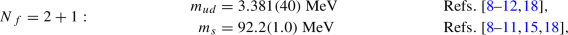(33)

and the RGI values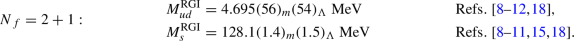(34)

$$N_{\,f}=2+1+1$$ lattice calculations

Since the previous review a new computation of $$m_s, m_{ud}$$ has appeared, ETM 21A . Using twisted-mass fermions with an added clover-term to suppress $${\mathcal {O}}(a^2)$$ effects between the neutral and charged pions, this work represents a significant improvement over ETM 14 . Renormalization is performed nonperturbatively in the RI-MOM scheme. Their ensembles comprise three lattice spacings (0.095, 0.082, and 0.069 fm), two volumes for the finest lattice spacings with pion masses reaching down to the physical point in the two finest lattices allowing a controlled chiral extrapolation. Their volumes are large, with $$m_\pi L$$ between four and five. These characteristics of their ensembles pass the most stringent FLAG criteria in all categories. This work extracts quark masses from two different quantities, one based on the meson spectrum and the other based on the baryon spectrum. Results obtained with these two methods agree within errors. The latter agrees well with the FLAG average while the former is high in comparison (there is good agreement with their previous results, ETM 14 ). Since ETM 21A was not published by the FLAG deadline, it is not included in the averages.

There are three other works that enter in light-quark mass averages: FNAL/MILC/TUMQCD 18  (which contributes both to the average of $$m_{ud}$$ and $$m_s$$), and the $$m_{ud}$$ determinations in HPQCD 18  and HPQCD 14A .

While the results of HPQCD 14A and HPQCD 18 agree well (using different methods), there are several tensions in the determination of $$m_s$$. The most significant discrepancy is between ETM 21A and the FLAG average. But also two recent and very precise determinations (HPQCD 18 and FNAL/MILC/TUMQCD 18) show a tension. Overall there is a rough agreement between the different determinations with $$\chi ^2/\textrm{dof} = 1.7$$ (that we apply to our average according to the standard FLAG averaging procedure). In the case of $$m_{ud}$$ on the other hand only two works contribute to the average: ETM 14 and FNAL/MILC/TUMQCD 18. They disagree, with the FNAL/MILC/TUMQCD 18 value basically matching the $$N_{f}=2+1$$ result. The large $$\chi ^2/\textrm{dof} \approx 1.7$$ increases significantly the error of the average. These large values of the $$\chi ^2$$ are difficult to understand in terms of a statistical fluctuation. On the other hand the $$N_{f}=2+1$$ and $$N_{f}=2+1+1$$ averages show a good agreement, which increases our confidence in the averages quoted below.

The $$N_{\,f}=2+1+1$$ results are summarized in Table 7. Note that the results of Ref.  are reported as $$m_s(2\,\textrm{GeV};N_f=3)$$ and those of Ref.  as $$m_{ud(s)}(2\,\textrm{GeV};N_f=4)$$. We convert the former to $$N_f=4$$ and obtain $$m_s(2\,\textrm{GeV};N_f=4)=93.7(8)\textrm{MeV}$$. The average of FNAL/MILC/TUMQCD 18, HPQCD 18, ETM 14 and HPQCD 14A is $$93.43(70)\textrm{MeV}$$ with $$\chi ^2/\hbox {dof}=1.7$$. For the light-quark average we use ETM 14 and FNAL/MILC/TUMQCD 18 with an average $$3.410(43)\textrm{MeV}$$ and a $$\chi ^2/\hbox {dof}=1.7$$. We note these $$\chi ^2$$ values are large. For the case of the light-quark masses this is mostly due to ETM 14 masses lying significantly above the rest, but in the case of $$m_s$$ there is also some tension between the recent and very precise results of HPQCD 18 and FNAL/MILC/TUMQCD 18. Also note that the 2+1-flavour values are consistent with the four-flavour ones, so in all cases we have decided to simply quote averages according to FLAG rules, including stretching factors for the errors based on $$\chi ^2$$ values of our fits: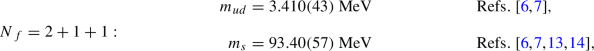(35)

and the RGI values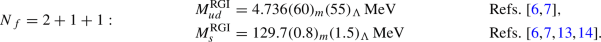(36)

In Figs. 1 and 2 the lattice results listed in Tables 6 and 7 and the FLAG averages obtained at each value of $$N_f$$ are presented and compared with various phenomenological results.

#### 3.1.5 Lattice determinations of $$m_s/m_{ud}$$

The lattice results for $$m_s/m_{ud}$$ are summarized in Table 8. In the ratio $$m_s/m_{ud}$$, one of the sources of systematic error – the uncertainties in the renormalization factors – drops out. Also other systematic effects (like the effect of the scale setting) are reduced in these ratios. This might explain that despite the discrepancies that are present in the individual quark mass determinations, the ratios show an overall very good agreement.

$$N_{\,f}=2+1$$ lattice calculations

ALPHA 19 , discussed already, is the only new result for this section. The other works contributing to this average are RBC/UKQCD 14B, which replaces RBC/UKQCD 12 (see Sect. 3.1.4), and the results of MILC 09A and BMW 10A, 10B.

The results show very good agreement with a $$\chi ^2/\textrm{dof} = 0.14$$. The final uncertainty ($$\approx 0.5\%$$) is smaller than the ones of the quark masses themselves. At this level of precision, the uncertainties in the electromagnetic and strong isospin-breaking corrections might not be completely negligible. Nevertheless, we decided not to add any uncertainty associated with this effect. The main reason is that most recent determinations try to estimate this uncertainty themselves and found an effect smaller than naive power counting estimates (see $$N_{\,f}=2+1+1$$ section),

\begin{aligned} N_f = 2+1 : \qquad {m_s}/{m_{ud}} = 27.42 ~ (12) \qquad \,\mathrm {Refs.}~ [8-10, 15, 18]. \end{aligned}
(37)

$$N_{\,f}=2+1+1$$ lattice calculations

For $$N_f = 2+1+1$$ there are three results, MILC 17 , ETM 14  and FNAL/MILC 14A , all of which satisfy our selection criteria.

All these works have been discussed in the previous FLAG edition , except the new result ETM 21A, that we have already examined (and anyway does not appear in the average because it was unpublished at the deadline). The fit has $$\chi ^2/\textrm{dof} \approx 2.5$$, and the result shows reasonable agreement with the $$N_{f}=2+1$$ result.

\begin{aligned} N_f = 2+1+1 :\qquad m_s / m_{ud} = 27.23 ~ (10)\qquad \,\mathrm {Refs.}~[7, 16, 17], \end{aligned}
(38)

which corresponds to an overall uncertainty equal to 0.4%. It is worth noting that  estimates the EM effects in this quantity to be $$\sim 0.18\%$$ (or 0.049 which is less than the quoted error above).

All the lattice results listed in Table 8 as well as the FLAG averages for each value of $$N_f$$ are reported in Fig. 3 and compared with $$\chi \hbox {PT}$$ and sum rules.

#### 3.1.6 Lattice determination of $$m_u$$ and $$m_d$$

In addition to reviewing computations of individual $$m_u$$ and $$m_d$$ quark masses, we will also determine FLAG averages for the parameter $$\epsilon$$ related to the violations of Dashen’s theorem

\begin{aligned} \epsilon =\frac{(\Delta M_{K}^{2}-\Delta M_{\pi }^{2})^{\gamma }}{\Delta M_{\pi }^{2}}, \end{aligned}
(39)

where $$\Delta M_{\pi }^{2}=M_{\pi ^+}^{2}-M_{\pi ^0}^{2}$$ and $$\Delta M_{K}^{2}=M_{K^+}^{2}-M_{K^0}^{2}$$ are the pion and kaon squared mass splittings, respectively. The superscript $$\gamma$$, here and in the following, denotes corrections that arise from electromagnetic effects only. This parameter is often a crucial intermediate quantity in the extraction of the individual light-quark masses. Indeed, it can be shown, using the G-parity symmetry of the pion triplet, that $$\Delta M_{\pi }^{2}$$ does not receive $${\mathcal {O}}(\delta m)$$ isospin-breaking corrections. In other words

\begin{aligned} \Delta M_{\pi }^{2}=(\Delta M_{\pi }^{2})^{\gamma } \qquad \text {and} \qquad \epsilon =\frac{(\Delta M_{K}^{2})^{\gamma }}{\Delta M_{\pi }^{2}}-1, \end{aligned}
(40)

at leading-order in the isospin-breaking expansion. The difference $$(\Delta M_{\pi }^{2})^{SU(2)}$$ was estimated in previous editions of FLAG through the $$\epsilon _m$$ parameter. However, consistent with our leading-order truncation of the isospin-breaking expansion, it is simpler to ignore this term. Once known, $$\epsilon$$ allows one to consistently subtract the electromagnetic part of the kaon-mass splitting to obtain the QCD splitting $$(\Delta M_{K}^{2})^{SU(2)}$$. In contrast with the pion, the kaon QCD splitting is sensitive to $$\delta m$$, and, in particular, proportional to it at leading order in $$\chi$$PT. Therefore, the knowledge of $$\epsilon$$ allows for the determination of $$\delta m$$ from a chiral fit to lattice-QCD data. Originally introduced in another form in , $$\epsilon$$ vanishes in the SU(3) chiral limit, a result known as Dashen’s theorem. However, in the 1990s numerous phenomenological papers pointed out that $$\epsilon$$ might be an $${\mathcal {O}}(1)$$ number, indicating a significant failure of SU(3) $$\chi$$PT in the description of electromagnetic effects on light-meson masses. However, the phenomenological determinations of $$\epsilon$$ feature some level of controversy, leading to the rather imprecise estimate $$\epsilon =0.7(5)$$ given in the first edition of FLAG. Starting with the FLAG 19 edition of the review, we quote more precise averages for $$\epsilon$$, directly obtained from lattice-QCD+QED simulations. We refer the reader to earlier editions of FLAG and to the review  for discussions of the phenomenological determinations of $$\epsilon$$.

The quality criteria regarding finite-volume effects for calculations including QED are presented in Sect. 2.1.1. Due to the long-distance nature of the electromagnetic interaction, these effects are dominated by a power law in the lattice spatial size. The coefficients of this expansion depend on the chosen finite-volume formulation of QED. For $$\textrm{QED}_{\textrm{L}}$$, these effects on the squared mass $$M^2$$ of a charged meson are given by [147, 148, 150]

\begin{aligned} \Delta _{\textrm{FV}}M^2= \alpha M^2\left\{ \frac{c_{1}}{ML}+\frac{2c_1}{(ML)^2}+ {\mathcal {O}}\left[ \frac{1}{(ML)^3}\right] \right\} \,,\end{aligned}
(41)

with $$c_1\simeq -2.83730$$. It has been shown in  that the two first orders in this expansion are exactly known for hadrons, and are equal to the pointlike case. However, the $${\mathcal {O}}[1/(ML)^{3}]$$ term and higher orders depend on the structure of the hadron. The universal corrections for $$\textrm{QED}_{\textrm{TL}}$$ can also be found in . In all this part, for all computations using such universal formulae, the QED finite-volume quality criterion has been applied with $$n_{\textrm{min}}=3$$, otherwise $$n_{\textrm{min}}=1$$ was used.

Since FLAG 19, six new results have been reported for nondegenerate light-quark masses. In the $$N_f=2+1+1$$ sector, MILC 18  computed $$\epsilon$$ using $$N_f=2+1$$ asqtad electro-quenched QCD+$$\textrm{QED}_{\textrm{TL}}$$ simulations and extracted the ratio $$m_u/m_d$$ from a new set of $$N_f=2+1+1$$ HISQ QCD simulations. Although $$\epsilon$$ comes from $$N_f=2+1$$ simulations, $$(\Delta M_{K}^{2})^{SU(2)}$$, which is about three times larger than $$(\Delta M_{K}^{2})^{\gamma }$$, has been determined in the $$N_f=2+1+1$$ theory. We therefore chose to classify this result as a four-flavour one. This result is explicitly described by the authors as an update of MILC 17 . In MILC 17 , $$m_u/m_d$$ is determined as a side-product of a global analysis of heavy-meson decay constants, using a preliminary version of $$\epsilon$$ from MILC 18 . In FNAL/MILC/TUMQCD 18  the ratio $$m_u/m_d$$ from MILC 17  is used to determine the individual masses $$m_u$$ and $$m_d$$ from a new calculation of $$m_{ud}$$. The work RM123 17  is the continuation of the $$N_f=2$$ work named RM123 13  in the previous edition of FLAG. This group now uses $$N_f=2+1+1$$ ensembles from ETM 10 , however, still with a rather large minimum pion mass of $$270~{\textrm{MeV}}$$, leading to therating for chiral extrapolations. In the $$N_f=2+1$$ sector, BMW 16  reuses the data set produced from their determination of the light-baryon octet-mass splittings  using electro-quenched QCD+$$\textrm{QED}_{\textrm{TL}}$$ smeared clover fermion simulations. Finally, MILC 16 , which is a preliminary result for the value of $$\epsilon$$ published in MILC 18 , also provides a $$N_f=2+1$$ computation of the ratio $$m_u/m_d$$.

MILC 09A  uses the mass difference between $$K^0$$ and $$K^+$$, from which they subtract electromagnetic effects using Dashen’s theorem with corrections, as discussed in the introduction of this section. The up and down sea quarks remain degenerate in their calculation, fixed to the value of $$m_{ud}$$ obtained from $$M_{\pi ^0}$$. To determine $$m_u/m_d$$, BMW 10A, 10B [9, 10] follow a slightly different strategy. They obtain this ratio from their result for $$m_s/m_{ud}$$ combined with a phenomenological determination of the isospin-breaking quark-mass ratio $$Q=22.3(8)$$, from $$\eta \rightarrow 3\pi$$ decays  (the decay $$\eta \rightarrow 3\pi$$ is very sensitive to QCD isospin breaking, but fairly insensitive to QED isospin breaking). Instead of subtracting electromagnetic effects using phenomenology, RBC 07  and Blum 10  actually include a quenched electromagnetic field in their calculation. This means that their results include corrections to Dashen’s theorem, albeit only in the presence of quenched electromagnetism. Since the up and down quarks in the sea are treated as degenerate, very small isospin corrections are neglected, as in MILC’s calculation. PACS-CS 12  takes the inclusion of isospin-breaking effects one step further. Using reweighting techniques, it also includes electromagnetic and $$m_u-m_d$$ effects in the sea. However, they do not correct for the large finite-volume effects coming from electromagnetism in their $$M_{\pi }L\sim 2$$ simulations, but provide rough estimates for their size, based on Ref. . QCDSF/UKQCD 15  uses QCD+QED dynamical simulations performed at the SU(3)-flavour-symmetric point, but at a single lattice spacing, so they do not enter our average. The smallest partially quenched ($$m_\textrm{sea}\ne m_\textrm{val}$$) pion mass is greater than 200 MeV, so our chiral-extrapolation criteria require arating. Concerning finite-volume effects, this work uses three spatial extents L of $$1.6~\textrm{fm}$$, $$2.2~\textrm{fm}$$, and $$3.3~\textrm{fm}$$. QCDSF/UKQCD 15 claims that the volume dependence is not visible on the two largest volumes, leading them to assume that finite-size effects are under control. As a consequence of that, the final result for quark masses does not feature a finite-volume extrapolation or an estimation of the finite-volume uncertainty. However, in their work on the QED corrections to the hadron spectrum  based on the same ensembles, a volume study shows some level of compatibility with the $$\textrm{QED}_{\textrm{L}}$$ finite-volume effects derived in . We see two issues here. Firstly, the analytical result quoted from  predicts large, $${\mathcal {O}}(10\%)$$ finite-size effects from QED on the meson masses at the values of $$M_{\pi }L$$ considered in QCDSF/UKQCD 15, which is inconsistent with the statement made in the paper. Secondly, it is not known that the zero-mode regularization scheme used here has the same volume scaling as $$\textrm{QED}_{\textrm{L}}$$. We therefore chose to assign therating for finite volume to QCDSF/UKQCD 15. Finally, for $$N_f=2+1+1$$, ETM 14  uses simulations in pure QCD, but determines $$m_u-m_d$$ from the slope $$\partial M_K^2/\partial m_{ud}$$ and the physical value for the QCD kaon-mass splitting taken from the phenomenological estimate in FLAG 13.

Lattice results for $$m_u$$, $$m_d$$ and $$m_u/m_d$$ are summarized in Table 9. The colour coding is specified in detail in Sect. 2.1. Considering the important progress in the last years on including isospin-breaking effects in lattice simulations, we are now in a position where averages for $$m_u$$ and $$m_d$$ can be made without the need of phenomenological inputs. Therefore, lattice calculations of the individual quark masses using phenomenological inputs for isospin-breaking effects will be coded.

We start by recalling the $$N_f=2$$ FLAG average for the light-quark masses, entirely coming from RM123 13 ,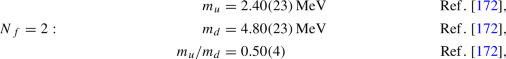(42)

with errors of roughly 10%, 5% and 8%, respectively. In these results, the errors are obtained by combining the lattice statistical and systematic errors in quadrature. For $$N_{\,f}=2+1$$, the only result, which qualifies for entering the FLAG average for quark masses, is BMW 16 ,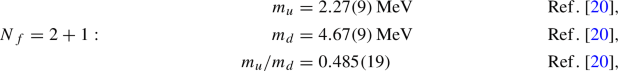(43)

with errors of roughly 4%, 2% and 4%, respectively. This estimate is slightly more precise than in the previous edition of FLAG. More importantly, it now comes entirely from a lattice-QCD+QED calculation, whereas phenomenological input was used in previous editions. These numbers result in the following RGI averages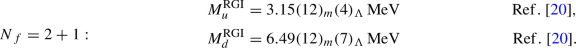(44)

Finally, for $$N_{\,f}=2+1+1$$, RM123 17  and FNAL/MILC/TUMQCD 18  enter the average for the individual $$m_u$$ and $$m_d$$ masses, and RM123 17  and MILC 18  enter the average for the ratio $$m_u/m_d$$, giving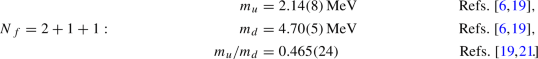(45)

with errors of roughly 4%, 1% and 5%, respectively. One can observe some marginal discrepancies between results coming from the MILC collaboration and RM123 17 . More specifically, adding all sources of uncertainties in quadrature, one obtains a 1.7$$\sigma$$ discrepancy between RM123 17  and MILC 18  for $$m_u/m_d$$, and a 2.2$$\sigma$$ discrepancy between RM123 17  and FNAL/MILC/TUMQCD 18  for $$m_u$$. However, the values of $$m_d$$ and $$\epsilon$$ are in very good agreement between the two groups. These discrepancies are presently too weak to constitute evidence for concern, and will be monitored as more lattice groups provide results for these quantities. The RGI averages for $$m_u$$ and $$m_d$$ are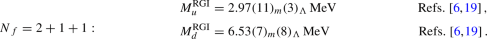(46)

Every result for $$m_u$$ and $$m_d$$ used here to produce the FLAG averages relies on electro-quenched calculations, so there is some interest to comment on the size of quenching effects. Considering phenomenology and the lattice results presented here, it is reasonable for a rough estimate to use the value $$(\Delta M_{K}^{2})^{\gamma }\sim 2000~{\textrm{MeV}}^2$$ for the QED part of the kaon-mass splitting. Using the arguments presented in Sect. 3.1.3, one can assume that the QED sea contribution represents $${\mathcal {O}}(10\%)$$ of $$(\Delta M_{K}^{2})^{\gamma }$$. Using SU(3) $$\hbox {PQ}\chi \hbox {PT+QED}$$ [174, 223] gives a $$\sim 5\%$$ effect. Keeping the more conservative $$10\%$$ estimate and using the experimental value of the kaon-mass splitting, one finds that the QCD kaon-mass splitting $$(\Delta M_{K}^{2})^{SU(2)}$$ suffers from a reduced $$3\%$$ quenching uncertainty. Considering that this splitting is proportional to $$m_u-m_d$$ at leading order in SU(3) $$\chi \hbox {PT}$$, we can estimate that a similar error will propagate to the quark masses. So the individual up and down masses look mildly affected by QED quenching. However, one notices that $$\sim 3\%$$ is the level of error in the new FLAG averages, and increasing significantly this accuracy will require using fully unquenched calculations.

In view of the fact that a massless up quark would solve the strong CP problem, many authors have considered this an attractive possibility, but the results presented above exclude this possibility: the value of $$m_u$$ in Eq. (43) differs from zero by 26 standard deviations. We conclude that nature solves the strong CP problem differently.

Finally, we conclude this section by giving the FLAG averages for $$\epsilon$$ defined in Eq. (39). For $$N_{\,f}=2+1+1$$, we average the results of RM123 17  and MILC 18  with the value of $$(\Delta M_{K}^{2})^{\gamma }$$ from BMW 14  combined with Eq. (40), giving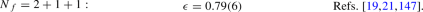(47)

Although BMW 14  focuses on hadron masses and did not extract the light-quark masses, they are the only fully unquenched QCD+QED calculation to date that qualifies to enter a FLAG average. With the exception of renormalization, which is not discussed in the paper, this work has arating for every FLAG criterion considered for the $$m_u$$ and $$m_d$$ quark masses. For $$N_{\,f}=2+1$$ we use the results from BMW 16 ,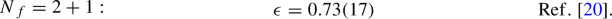(48)

It is important to notice that the $$\epsilon$$ uncertainties from BMW 16 and RM123 17 are dominated by estimates of the QED quenching effects. Indeed, in contrast with the quark masses, $$\epsilon$$ is expected to be rather sensitive to the sea-quark QED contributions. Using the arguments presented in Sect. 3.1.3, if one conservatively assumes that the QED sea contributions represent $${\mathcal {O}}(10\%)$$ of $$(\Delta M_{K}^{2})^{\gamma }$$, then Eq. (40) implies that $$\epsilon$$ will have a quenching error of $$\sim 0.15$$ for $$(\Delta M_{K}^{2})^{\gamma }\sim 2000~{\textrm{MeV}}^2$$, representing a large $$\sim 20\%$$ relative error. It is interesting to observe that such a discrepancy does not appear between BMW 15 and RM123 17, although the $$\sim 10\%$$ accuracy of both results might not be sufficient to resolve these effects. On the other hand, in the context of SU(3) chiral perturbation theory, Bijnens and Danielsson  show that the QED quenching effects on $$\epsilon$$ do not depend on unknown LECs at NLO and are therefore computable at that order. In that approach, MILC 18 finds the effect at NLO to be only 5%. To conclude, although the controversy around the value of $$\epsilon$$ has been significantly reduced by lattice-QCD+QED determinations, computing this at few-percent accuracy requires simulations with charged sea quarks.

#### 3.1.7 Estimates for R and Q

The quark-mass ratios

\begin{aligned} R\equiv \frac{m_s-m_{ud}}{m_d-m_u}\quad \hbox {and}\quad Q^2\equiv \frac{m_s^2-m_{ud}^2}{m_d^2-m_u^2} \end{aligned}
(49)

compare SU(3) breaking with isospin breaking. Both numbers only depend on the ratios $$m_s/m_{ud}$$ and $$m_u/m_d$$,

\begin{aligned} R=\frac{1}{2}\left( \frac{m_s}{m_{ud}}-1\right) \frac{1+\frac{m_u}{m_d}}{1-\frac{m_u}{m_d}} \qquad \text {and}\qquad Q^2=\frac{1}{2}\left( \frac{m_s}{m_{ud}}+1\right) R. \end{aligned}
(50)

The quantity Q is of particular interest because of a low-energy theorem , which relates it to a ratio of meson masses,

\begin{aligned} Q^2_M\equiv \frac{\hat{M}_K^2}{\hat{M}_\pi ^2}\frac{\hat{M}_K^2-\hat{M}_\pi ^2}{\hat{M}_{K^0}^2- \hat{M}_{K^+}^2}\,,\qquad \hat{M}^2_\pi \equiv \frac{1}{2}( \hat{M}^2_{\pi ^+}+ \hat{M}^2_{\pi ^0}) \,,\qquad \hat{M}^2_K\equiv \frac{1}{2}( \hat{M}^2_{K^+}+ \hat{M}^2_{K^0})\,.\end{aligned}
(51)

(We remind the reader that the $$\,\hat{ }\,$$ denotes a quantity evaluated in the $$\alpha \rightarrow 0$$ limit.) Chiral symmetry implies that the expansion of $$Q_M^2$$ in powers of the quark masses (i) starts with $$Q^2$$ and (ii) does not receive any contributions at NLO: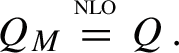(52)

We recall here the $$N_f=2$$ estimates for Q and R from FLAG 16,

\begin{aligned} R=40.7(3.7)(2.2)\,,\qquad Q=24.3(1.4)(0.6) ,\end{aligned}
(53)

where the second error comes from the phenomenological inputs that were used. For $$N_{\,f}=2+1$$, we use Eqs. (37) and (43) and obtain

\begin{aligned} R=38.1(1.5)\,,\qquad Q=23.3(0.5) ,\end{aligned}
(54)

where now only lattice results have been used. For $$N_{\,f}=2+1+1$$ we obtain

\begin{aligned} R=35.9(1.7)\,,\qquad Q=22.5(0.5) ,\end{aligned}
(55)

which are quite compatible with two- and three-flavour results. It is interesting to notice that the most recent phenomenological determination of R and Q from $$\eta \rightarrow 3\pi$$ decay  gives the values $$R=34.4(2.1)$$ and $$Q=22.1(7)$$, which are marginally discrepant with some of the averages presented here. The authors of [225, 226] point out that this discrepancy is likely due to surprisingly large corrections to the approximation in Eq. (52) used in the phenomenological analysis.

Our final results for the masses $$m_u$$, $$m_d$$, $$m_{ud}$$, $$m_s$$ and the mass ratios $$m_u/m_d$$, $$m_s/m_{ud}$$, R, Q are collected in Tables 10 and 11.

### 3.2 Charm-quark mass

In the following, we collect and discuss the lattice determinations of the $$\overline{\textrm{MS}}$$ charm-quark mass $$\overline{m}_c$$. Most of the results have been obtained by analyzing the lattice-QCD simulations of two-point heavy-light- or heavy-heavy-meson correlation functions, using as input the experimental values of the D, $$D_s$$, and charmonium mesons. Some groups use the moments method. The latter is based on the lattice calculation of the Euclidean time moments of pseudoscalar-pseudoscalar correlators for heavy-quark currents followed by an OPE expansion dominated by perturbative QCD effects, which provides the determination of both the heavy-quark mass and the strong-coupling constant $$\alpha _s$$.

The heavy-quark actions adopted by various lattice collaborations have been discussed in previous FLAG reviews [2,3,4], and their descriptions can be found in Sec. A.1.3 of FLAG 19 . While the charm mass determined with the moments method does not need any lattice evaluation of the mass-renormalization constant $$Z_m$$, the extraction of $$\overline{m}_c$$ from two-point heavy-meson correlators does require the nonperturbative calculation of $$Z_m$$. The lattice scale at which $$Z_m$$ is obtained is usually at least of the order 2–3 GeV, and therefore it is natural in this review to provide the values of $$\overline{m}_c(\mu )$$ at the renormalization scale $$\mu = 3~\textrm{GeV}$$. Since the choice of a renormalization scale equal to $$\overline{m}_c$$ is still commonly adopted (as by the PDG ), we have collected in Table 12 the lattice results for both $$\overline{m}_c(\overline{m}_c)$$ and $$\overline{m}_c(\hbox {3 GeV})$$, obtained for $$N_f =2+1$$ and $$2+1+1$$. For $$N_f=2$$, interested readers are referred to previous reviews [2, 3].

When not directly available in the published work, we apply a conversion factor using perturbative QCD evolution at five loops to run down from $$\mu = 3$$ GeV to the scales $$\mu = \overline{m}_c$$ and 2 GeV of 0.7739(60) and 0.9026(23), respectively, where the error comes from the uncertainty in $$\Lambda _\textrm{QCD}$$. We use $$\Lambda _\textrm{QCD} = 297(12)$$ MeV for $$N_f = 4$$ (see Sect. 9). Perturbation theory uncertainties, estimated as the difference between results that use 4- and 5-loop running, are significantly smaller than the parametric uncertainty coming from $$\Lambda _\textrm{QCD}$$. For $$\mu = \overline{m}_c$$, the former is about about 2.5 times smaller. Given the high precision of many of these results, future works should take the uncertainties in $$\Lambda _\textrm{QCD}$$ and perturbation theory seriously.

In the next subsections we review separately the results for $$\overline{m}_c$$ with three or four flavours of quarks in the sea.

#### 3.2.1 $$N_f = 2+1$$ results

Since the last review , there are two new results, Petreczky 19  and ALPHA 21 , the latter of which was not published at the FLAG deadline. Petreczky 19 employs the HISQ action on ten ensembles with ten lattice spacings down to 0.025 fm, physical strange-quark mass, and two light-quark masses, the lightest corresponding to 161 MeV pions. Their study incorporates lattices with 11 different sizes, ranging from 1.6 to 5.4 fm. The masses are computed from moments of pseudoscalar quarkonium correlation functions, and $$\overline{\textrm{MS}}$$ masses are extracted with 4-loop continuum perturbation theory. Thus this work easily rates green stars in all categories. ALPHA 21 uses the $${\mathcal {O}}(a)$$-improved Wilson-clover action with five lattice spacings from 0.087 to 0.039 fm, produced by the CLS collaboration. For each lattice spacing, several light sea-quark masses are used in a global chiral-continuum extrapolation (the lightest pion mass for one ensemble is 198 MeV). The authors also use nonperturbative renormalization and running through application of step-scaling and the Schrödinger functional scheme. Finite-volume effects are investigated at one lattice spacing and only for $$\sim 400$$ MeV pions on the smallest two volumes where results are compatible within statistical errors. ALPHA 21 satisfies the FLAG criteria for green-star ratings in all of the categories listed in Table 12, but because it is a new result that was unpublished at the deadline, does not enter the average in this review.

Descriptions of the other works in this section can be found in the last review .

According to our rules on the publication status, the FLAG average for the charm-quark mass at $$N_f = 2+1$$ is obtained by combining the results HPQCD 10, $$\chi$$QCD 14, JLQCD 16, and Petreczky 19,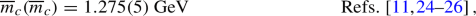(56)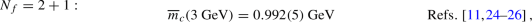(57)

where the error on $$\overline{m}_c(\overline{m}_c)$$ includes a stretching factor $$\sqrt{\chi ^2/\hbox {dof}} \simeq 1.16$$ as discussed in Sect. 2.2. This result corresponds to the following RGI average

\begin{aligned}{} & {} M_c^\textrm{RGI}&= 1.526(9)_m(14)_\Lambda ~ \textrm{GeV}{} & {} \,\mathrm {Refs.}~[11, 24-26]. \end{aligned}
(58)

#### 3.2.2 $$N_f = 2+1+1$$ results

For a discussion of older results, see the previous FLAG reviews. Since FLAG 19 two groups have produced updated values with charm quarks in the sea.

HPQCD 20A  is an update of HPQCD 18, including a new finer ensemble ($$a\approx 0.045$$ fm) and EM corrections computed in the quenched approximation of QED for the first time. Besides these new items, the analysis is largely unchanged from HPQCD 18 except for an added $$\alpha _s^3$$ correction to the SMOM-to-$$\overline{\textrm{MS}}$$ conversion factor and tuning the bare charm mass via the $$J/\psi$$ mass rather than the $$\eta _c$$. Their new value in pure QCD is $$\overline{m}_c(3~\textrm{GeV})=0.9858(51)$$ GeV which is quite consistent with HPQCD 18 and the FLAG 19 average. The effects of quenched QED in both the bare charm-quark mass and the renormalization constant are small. Both effects are precisely determined, and the overall effect shifts the mass down slightly to $$\overline{m}_c(3~\textrm{GeV})=0.9841(51)$$ where the uncertainty due to QED is invisible in the final error. The shift from their pure QCD value due to quenched QED is about $$-0.2\%$$.

ETM 21A  is a new work that follows a similar methodology as ETM 14, but with significant improvements. Notably, a clover-term is added to the twisted mass fermion action which suppresses $${\mathcal {O}}(a^2)$$ effects between the neutral and charged pions. Additional improvements include new ensembles lying very close to the physical mass point, better control of nonperturbative renormalization systematics, and use of both meson and baryon correlation functions to determine the quark mass. They use the RI-MOM scheme for nonperturbative renormalization. The analysis comprises ten ensembles in total with three lattice spacings (0.095, 0.082, and 0.069 fm), two volumes for the finest lattice spacings and four for the other two, and pion masses down to 134 MeV for the finest ensemble. The values of $$m_\pi L$$ range mostly from almost four to greater than five. According to the FLAG criteria, green stars are earned in all categories. The authors find $$m_c(3~\mathrm GeV)=1.036(17)(^{+15}_{-8})$$ GeV. In Table 12 we have applied a factor of 0.7739(60) to run from 3 GeV to $$\overline{m}_c$$. As in FLAG 19, the new value is consistent with ETM 14 and ETM 14A, but is still high compared to the FLAG average. The authors plan future improvements, including a finer lattice spacing for better control of the continuum limit and a new renormalization scheme, like RI-SMOM. This result has not been published by the deadline, so it does not yet appear in the average.

Five results enter the FLAG average for $$N_f = 2+1+1$$ quark flavours: ETM 14, ETM 14A, HPQCD 14A, FNAL/MILC/TUMQCD 18, and HPQCD 20A. We note that while the determinations of $$\overline{m}_c$$ by ETM 14 and 14A agree well with each other, they are incompatible with HPQCD 14A, FNAL/MILC/TUMQCD 18, and HPQCD 20A by several standard deviations. While the latter use the same configurations, the analyses are quite different and independent. As mentioned earlier, $$m_{ud}$$ and $$m_s$$ values by ETM are also systematically high compared to their respective averages. Combining all four results yields(59)(60)

where the errors include large stretching factors $$\sqrt{\chi ^2/\hbox {dof}}\approx 2.0$$ and 2.5, respectively. We have assumed 100% correlation for statistical errors between ETM results and the same for HPQCD 14A, HPQCD 20A, and FNAL/MILC/TUMQCD 18.

These are obviously poor $$\chi ^2$$ values, and the stretching factors are quite large. While it may be prudent in such a case to quote a range of values covering the central values of all results that pass the quality criteria, we believe in this case that would obscure rather than clarify the situation. From Fig. 5 we note that not only do ETM 21A, ETM 14A, and ETM 14 lie well above the other 2+1+1 results, but also above all of the 2+1 flavour results. A similar trend is apparent for the light-quark masses (see Figs. 1 and 2) while for mass ratios there is better agreement (Figs. 34 and 6). The latter suggests there may be underestimated systematic uncertainties associated with scale setting and/or renormalization which have not been detected. Finally we note the ETM results are significantly higher than the PDG average. For these reasons, which admittedly are not entirely satisfactory, we continue to quote an average with a stretching factor as in previous reviews.

The RGI average reads as follows,

\begin{aligned}{} & {} M_c^\textrm{RGI}&= 1.520(17)_m(14)_\Lambda ~ \textrm{GeV}{} & {} \,\mathrm {Refs.}~[6, 7, 14, 22, 23]. \end{aligned}
(61)

Figure 5 presents the values of $$\overline{m}_c(\overline{m}_c)$$ given in Table 12 along with the FLAG averages obtained for $$2+1$$ and $$2+1+1$$ flavours.

#### 3.2.3 Lattice determinations of the ratio $$m_c/m_s$$

Because some of the results for quark masses given in this review are obtained via the quark-mass ratio $$m_c/m_s$$, we review these lattice calculations, which are listed in Table 13, as well.

The $$N_f = 2+1$$ results from $$\chi \hbox {QCD}$$ 14 and HPQCD 09A  are from the same calculations that were described for the charm-quark mass in the previous review. Maezawa 16 does not pass our chiral-limit test (see the previous review), though we note that it is quite consistent with the other values. Combining $$\chi \hbox {QCD}$$ 14 and HPQCD 09A, we obtain the same result reported in FLAG 19,

\begin{aligned} N_f = 2+1: \qquad m_c / m_s = 11.82(16)\qquad \,\mathrm {Refs.}~[24, 27], \end{aligned}
(62)

with a $$\chi ^2/\hbox {dof} \simeq 0.85$$.

Turning to $$N_f = 2+1+1$$, there is a new result from ETM 21A. The errors have actually increased compared to ETM 14, due to larger uncertainties in the baryon sector which enter their average with the meson sector. Again, ETM 21A does not yet enter the average since it was not published by the deadline for the review. See the earlier reviews for a discussion of previous results.

We note that some tension exists between the HPQCD 14A and FNAL/MILC/TUMQCD results. Combining these with ETM 14 yields

\begin{aligned} N_f = 2+1+1: \qquad m_c / m_s = 11.768(34)\qquad \,\mathrm {Refs.}~[6, 7, 14], \end{aligned}
(63)

where the error includes the stretching factor $$\sqrt{\chi ^2/\hbox {dof}} \simeq 1.5$$. We have assumed a 100% correlation of statistical errors for FNAL/MILC/TUMQCD 18 and HPQCD 14A.

Results for $$m_c/m_s$$ are shown in Fig. 6 together with the FLAG averages for $$N_f = 2+1$$ and $$2+1+1$$ flavours.

### 3.3 Bottom-quark mass

Now we review the lattice results for the $$\overline{\textrm{MS}}$$ bottom-quark mass $$\overline{m}_b$$. Related heavy-quark actions and observables have been discussed in previous FLAG reviews [2,3,4], and descriptions can be found in Sec. A.1.3 of FLAG 19 . In Table 14 we collect results for $$\overline{m}_b(\overline{m}_b)$$ obtained with $$N_f =2+1$$ and $$2+1+1$$ sea-quark flavours. Available results for the quark-mass ratio $$m_b / m_c$$ are also reported. After discussing the new results we evaluate the corresponding FLAG averages.

#### 3.3.1 $$N_f=2+1$$

There is one new three-flavour result since the last review, Petreczky 19, which was described already in the charm-quark section. The new result rates green stars, so our new average with HPQCD 10 is (both works quote values in the $$N_f = 5$$ theory, so we simply use those values),

\begin{aligned}&N_f= 2+1 :&\overline{m}_b(\overline{m}_b)&= 4.171 (20) ~ \textrm{GeV}{} & {} \,\mathrm {Refs.}~[11, 26]. \end{aligned}
(64)

The corresponding four-flavour RGI average is

\begin{aligned}&N_f= 2+1 :&M_b^\textrm{RGI}&= 6.881(33)_m(54)_\Lambda ~ \textrm{GeV}{} & {} \,\mathrm {Refs.}~[11, 26]. \end{aligned}
(65)

#### 3.3.2 $$N_f=2+1+1$$

HPQCD 21  is an update of HPQCD 14A (and replaces it in our average), including EM corrections for the first time for the b-quark mass. Four flavours of HISQ quarks are used on MILC ensembles with lattice spacings from about 0.09–0.03 fm. Ensembles with physical and unphysical mass sea-quarks are used. Quenched QED is used to obtain the dominant $${\mathcal {O}}(\alpha )$$ effect. The ratio of bottom- to charm-quark masses is computed in a completely nonperturbative formulation, and the b-quark mass is extracted using the value of $$\overline{m}_c(3~\textrm{GeV})$$ from HPQCD 20A. Since EM effects are included, the QED renormalization scale enters the ratio which is quoted for 3 GeV and $$N_f=4$$. The total error on the new result is more than two times smaller than for HPQCD 14A, but is only slightly smaller compared to the NRQCD result reported in HPQCD 14B. The inclusion of QED shifts the ratio $$m_b/m_c$$ up slightly from the pure QCD value by about one standard deviation, and the value of $$\overline{m}_b(m_b)$$ is consistent, within errors, to the other pure QCD results entering our average. Therefore we quote a single average.

HPQCD 14B employs the NRQCD action  to treat the b quark. The b-quark mass is computed with the moments method, that is, from Euclidean-time moments of two-point, heavy-heavy-meson correlation functions (see also Sect. 9.8 for a description of the method).

In HPQCD 14B the b-quark mass is computed from ratios of the moments $$R_n$$ of heavy current-current correlation functions, namely,

\begin{aligned} \left[ \frac{R_n r_{n-2}}{R_{n-2}r_n}\right] ^{1/2} \frac{\bar{M}_\textrm{kin}}{2 m_b} = \frac{\bar{M}_{\Upsilon ,\eta _b}}{2 {\bar{m}}_b(\mu )} ~ , \end{aligned}
(66)

where $$r_n$$ are the perturbative moments calculated at N$$^3$$LO, $$\bar{M}_\textrm{kin}$$ is the spin-averaged kinetic mass of the heavy-heavy vector and pseudoscalar mesons and $$\bar{M}_{\Upsilon ,\eta _b}$$ is the experimental spin average of the $$\Upsilon$$ and $$\eta _b$$ masses. The average kinetic mass $$\bar{M}_\textrm{kin}$$ is chosen since in the lattice calculation the splitting of the $$\Upsilon$$ and $$\eta _b$$ states is inverted. In Eq. (66), the bare mass $$m_b$$ appearing on the left-hand side is tuned so that the spin-averaged mass agrees with experiment, while the mass $$\overline{m}_b$$ at the fixed scale $$\mu = 4.18$$ GeV is extrapolated to the continuum limit using three HISQ (MILC) ensembles with $$a \approx$$ 0.15, 0.12 and 0.09 fm and two pion masses, one of which is the physical one. Their final result is $$\overline{m}_b(\mu = 4.18\, \textrm{GeV}) = 4.207(26)$$ GeV, where the error is from adding systematic uncertainties in quadrature only (statistical errors are smaller than $$0.1 \%$$ and ignored). The errors arise from renormalization, perturbation theory, lattice spacing, and NRQCD systematics. The finite-volume uncertainty is not estimated, but at the lowest pion mass they have $$m_\pi L \simeq 4$$, which leads to the tag.

The next four-flavour result  is from the ETM collaboration and updates their preliminary result appearing in a conference proceedings . The calculation is performed on a set of configurations generated with twisted-Wilson fermions with three lattice spacings in the range 0.06 – 0.09 fm and with pion masses in the range 210–440 MeV. The b-quark mass is determined from a ratio of heavy-light pseudoscalar meson masses designed to yield the quark pole mass in the static limit. The pole mass is related to the $$\overline{\textrm{MS}}$$ mass through perturbation theory at N$$^3$$LO. The key idea is that by taking ratios of ratios, the b-quark mass is accessible through fits to heavy-light(strange)-meson correlation functions computed on the lattice in the range $$\sim 1$$$$2\times m_c$$ and the static limit, the latter being exactly 1. By simulating below $$\overline{m}_b$$, taking the continuum limit is easier. They find $$\overline{m}_b(\overline{m}_b) = 4.26(3)(10)$$ GeV, where the first error is statistical and the second systematic. The dominant errors come from setting the lattice scale and fit systematics.

Gambino et al.  use twisted-mass-fermion ensembles from the ETM collaboration and the ETM ratio method as in ETM 16. Three values of the lattice spacing are used, ranging from 0.062 to 0.089 fm. Several volumes are also used. The light-quark masses produce pions with masses from 210 to 450 MeV. The main difference with ETM 16 is that the authors use the kinetic mass defined in the heavy-quark expansion (HQE) to extract the b-quark mass instead of the pole mass.

The final b-quark mass result is FNAL/MILC/TUM 18 . The mass is extracted from the same fit and analysis done for the charm quark mass. Note that relativistic HISQ valence masses reach the physical b mass on the two finest lattice spacings ($$a = 0.042$$ fm, 0.03 fm) at physical and 0.2 $$m_s$$ light-quark mass, respectively. In lattice units the heavy valence masses correspond to $$aM^\textrm{RGI} > 0.90$$, making the continuum extrapolation challenging, but the authors investigated the effect of leaving out the heaviest points from the fit, and the result did not noticeably change. Their results are also consistent with an analysis dropping the finest lattice from the fit. Since the b-quark mass region is only reached with two lattice spacings, we rate this work with a green circle for the continuum extrapolation. Note however that for other values of the quark masses they use up to five values of the lattice spacing (cf. their charm-quark mass determination).

All of the above results enter our average. We note that here the ETM 16 result is consistent with the average and a stretching factor on the error is not used. The average and error is dominated by the very precise FNAL/MILC/TUM 18 value,

\begin{aligned}&N_f= 2+1+1 :&\overline{m}_b(\overline{m}_b)&= 4.203 (11) ~ \textrm{GeV}{} & {} \,\mathrm {Refs.}~[6, 14, 28-31]. \end{aligned}
(67)

We have included a 100% correlation on the statistical errors of ETM 16 and Gambino 17, since the same ensembles are used in both. While FNAL/MILC/TUM 18 and HPQCD 21 also use the same MILC HISQ ensembles, the statistical error in the HPQCD 21 analysis is negligible, so we do not include a correlation between them. The average has $$\chi ^2/\textrm{dof}=0.02$$.

The above translates to the RGI average

\begin{aligned}&N_f= 2+1+1 :&M_b^\textrm{RGI}&= 6.934(18)_m(55)_\Lambda ~\textrm{GeV}{} & {} \,\mathrm {Refs.}~[6, 14, 28-31]. \end{aligned}
(68)

All the results for $$\overline{m}_b(\overline{m}_b)$$ discussed above are shown in Fig. 7 together with the FLAG averages corresponding to $$N_f=2+1$$ and $$2+1+1$$ quark flavours.

## 4 Leptonic and semileptonic kaon and pion decay and $$|V_{ud}|$$ and $$|V_{us}|$$

Authors: T. Kaneko, J. N. Simone, S. Simula, N. Tantalo

This section summarizes state-of-the-art lattice calculations of the leptonic kaon and pion decay constants and the kaon semileptonic-decay form factor and provides an analysis in view of the Standard Model. With respect to the previous edition of the FLAG review  the data in this section has been updated. As in Ref. , when combining lattice data with experimental results, we take into account the strong SU(2) isospin correction, either obtained in lattice calculations or estimated by using chiral perturbation theory ($$\chi$$PT), both for the kaon leptonic decay constant $$f_{K^\pm }$$ and for the ratio $$f_{K^\pm } / f_{\pi ^\pm }$$.

### 4.1 Experimental information concerning $$|V_{ud}|$$, $$|V_{us}|$$, $$f_+(0)$$ and $${f_{K^\pm }}/{f_{\pi ^\pm }}$$

The following review relies on the fact that precision experimental data on kaon decays very accurately determine the product $$|V_{us}|f_+(0)$$  and the ratio $$|V_{us}/V_{ud}|f_{K^\pm }/f_{\pi ^\pm }$$ [165, 232]:

\begin{aligned} |V_{us}| f_+(0) = 0.2165(4)\,, \; \left| \frac{V_{us}}{V_{ud}}\right| \frac{ f_{K^\pm }}{ f_{\pi ^\pm }} \; =0.2760(4)\,.\end{aligned}
(69)

Here and in the following, $$f_{K^\pm }$$ and $$f_{\pi ^\pm }$$ are the isospin-broken decay constants, respectively, in QCD. We will refer to the decay constants in the SU(2) isospin-symmetric limit as $$f_K$$ and $$f_\pi$$ (the latter at leading order in the mass difference ($$m_u - m_d$$) coincides with $$f_{\pi ^\pm }$$). The parameters $$|V_{ud}|$$ and $$|V_{us}|$$ are elements of the Cabibbo–Kobayashi–Maskawa matrix and $$f_+(q^2)$$ represents one of the form factors relevant for the semileptonic decay $$K^0\rightarrow \pi ^-\ell \,\nu$$, which depends on the momentum transfer q between the two mesons. What matters here is the value at $$q^2 = 0$$:. The pion and kaon decay constants are defined byFootnote 12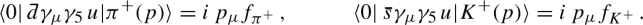In this normalization, $$f_{\pi ^\pm } \simeq 130$$ MeV, $$f_{K^\pm }\simeq 155$$ MeV.

In Eq. (69), the electromagnetic effects have already been subtracted in the experimental analysis using $$\chi$$PT. Recently, a new method  has been proposed for calculating the leptonic decay rates of hadrons including both QCD and QED on the lattice, and successfully applied to the case of the ratio of the leptonic decay rates of kaons and pions [239, 240]. The correction to the tree-level $$K_{\mu 2} / \pi _{\mu 2}$$ decay rate, including both electromagnetic and strong isospin-breaking effects, is found to be equal to $$-1.26 (14) \%$$Footnote 13 to be compared to the estimate $$-1.12 (21) \%$$ based on $$\chi$$PT [164, 241]. Using the experimental values of the $$K_{\mu 2}$$ and $$\pi _{\mu 2}$$ decay rates the result of Ref.  implies

\begin{aligned} \left| \frac{V_{us}}{V_{ud}}\right| \frac{f_K}{f_\pi } = 0.27683 \, (29)_\textrm{exp} \, (20)_\textrm{th} \,  ~ , \end{aligned}
(70)

where the last error in brackets is the sum in quadrature of the experimental and theoretical uncertainties, and the ratio of the decay constants is the one corresponding to isosymmetric QCD. A large part of the theoretical uncertainty comes from the statistics and continuum and chiral extrapolation of lattice data, which can be systematically reduced by a more realistic simulation with high statistics. We also note that an independent study of the electromagnetic effects is in progress . Therefore, it is feasible to more precisely determine $$|V_{us} / V_{ud}|$$ using only lattice-QCD+QED for $$f_{K^\pm } / f_{\pi ^\pm }$$ and the ratio of the experimental values of the $$K_{\mu 2}$$ and $$\pi _{\mu 2}$$ decay rates.

At present, the superallowed nuclear $$\beta$$ transitions provide the most precise determination of $$|V_{ud}|$$. Its accuracy has been limited by hadronic uncertainties in the universal electroweak radiative correction $$\Delta _R^V$$. A recent analysis in terms of a dispersion relation [243, 244] found $$\Delta _R^V$$ larger than the previous estimate . A more straightforward update of Ref.  also reported larger $$\Delta _R^V$$ . In the PDG review, the fourteen precisely measured transitions  with the dispersive estimate of $$\Delta _R^V$$ yield 

\begin{aligned} |V_{ud}| = 0.97370(14),\end{aligned}
(71)

which differs by $$\approx 3 \sigma$$ from the previous estimate . However, it is not a trivial matter to properly take account of the nuclear corrections at this precision [243, 247,248,249,250,251,252,253,254,255]. For example, the dispersive approach has been applied in a recent update of the so-called inner radiative correction due to quenching of the axial-vector and isoscalar spin-magnetic-moment couplings in nuclei , and in a recent estimate of a novel correction due to the distortion of the emitted electron energy spectrum by nuclear polarizabilities . A recent reanalysis of twenty-three $$\beta$$ decays  obtained

\begin{aligned} |V_{ud}| = 0.97373(31), \end{aligned}
(72)

where the two nuclear corrections tend to cancel with each other and, hence, leave the central value basically unchanged. Their uncertainties, however, doubles that of $$|V_{ud}|$$. In Sects. 4.4 and 4.5, we mainly use the PDG value (71) but also test Eq. (72) as an alternative input.

The matrix element $$|V_{us}|$$ can be determined from semi-inclusive $$\tau$$ decays [257,258,259,260]. By separating the inclusive decay $$\tau \rightarrow \hbox {hadrons}+\nu$$ into nonstrange and strange final states, e.g., HFLAV 18  obtains

\begin{aligned} |V_{us}| = 0.2195(19), \end{aligned}
(73)

and both Maltman et al. [259, 262, 263] and Gamiz et al. [264, 265] arrived at very similar values. Inclusive hadronic $$\tau$$ decay offers an interesting w